# RS Aggarwal Solutions for Class 9 Chapter 7: Lines and Angles Exercise 7C

Class 9 Maths introduces students to various new topics which are important from exam point of view. The students without knowledge about the concepts very often memorize the answers by heart. This leads to forgetting the steps while appearing for the exams. The objective of preparing solutions is to help students understand the methods of solving. While solving the problems from RS Aggarwal textbook, the students can make use of the PDF as a reference guide to perform well in exams. RS Aggarwal Solutions for Class 9 Maths Chapter 7 Lines and Angles Exercise 7C are provided here.

## RS Aggarwal Solutions for Class 9 Chapter 7: Lines and Angles Exercise 7C Download PDF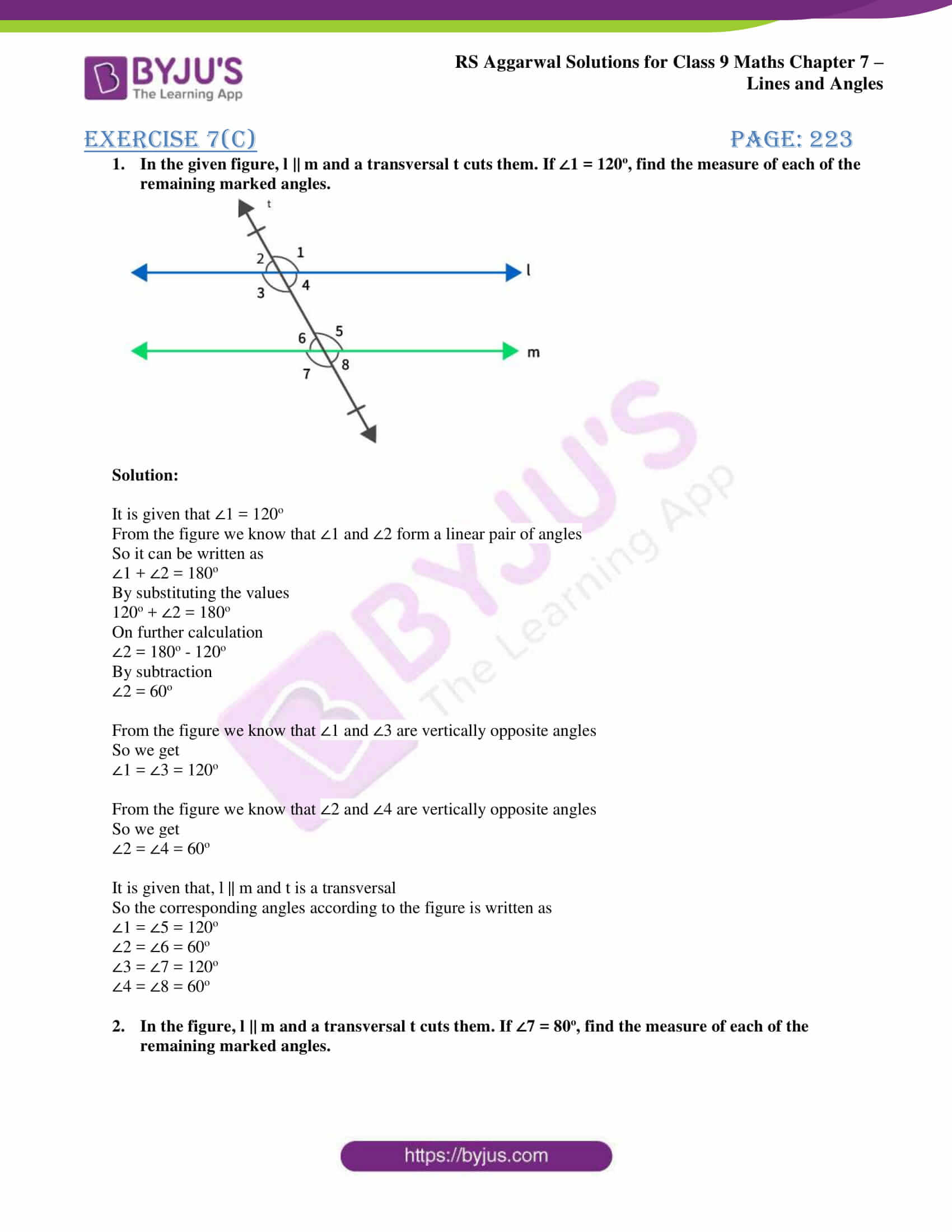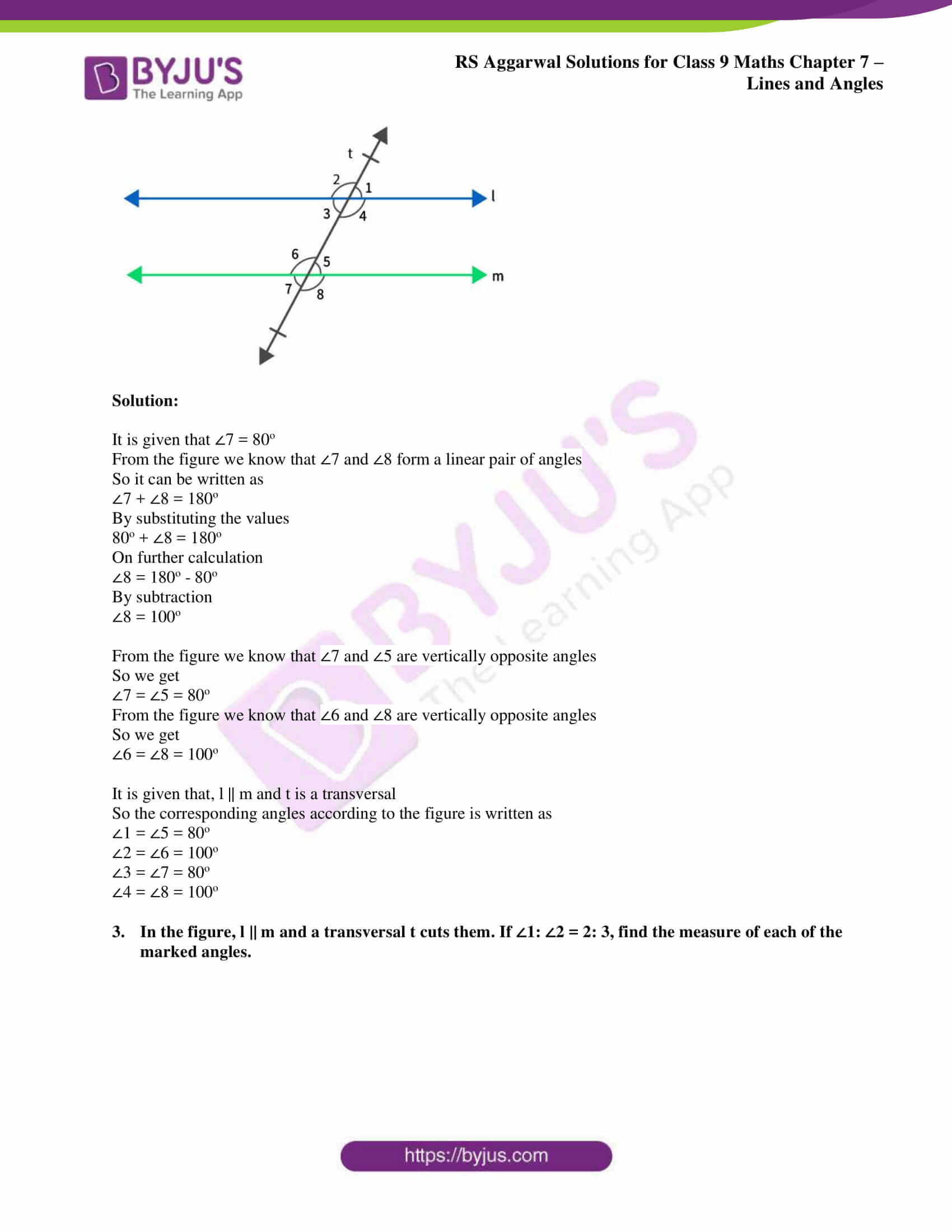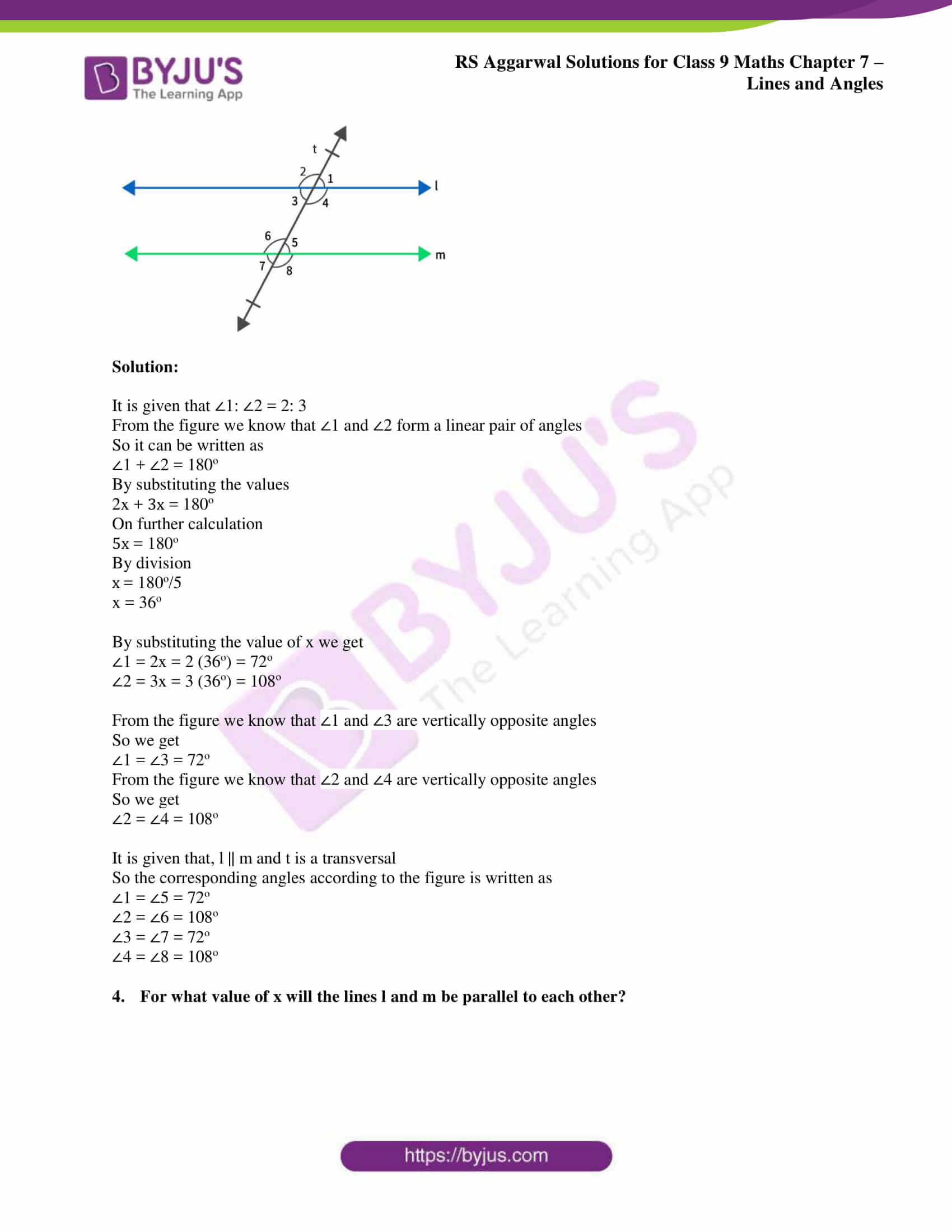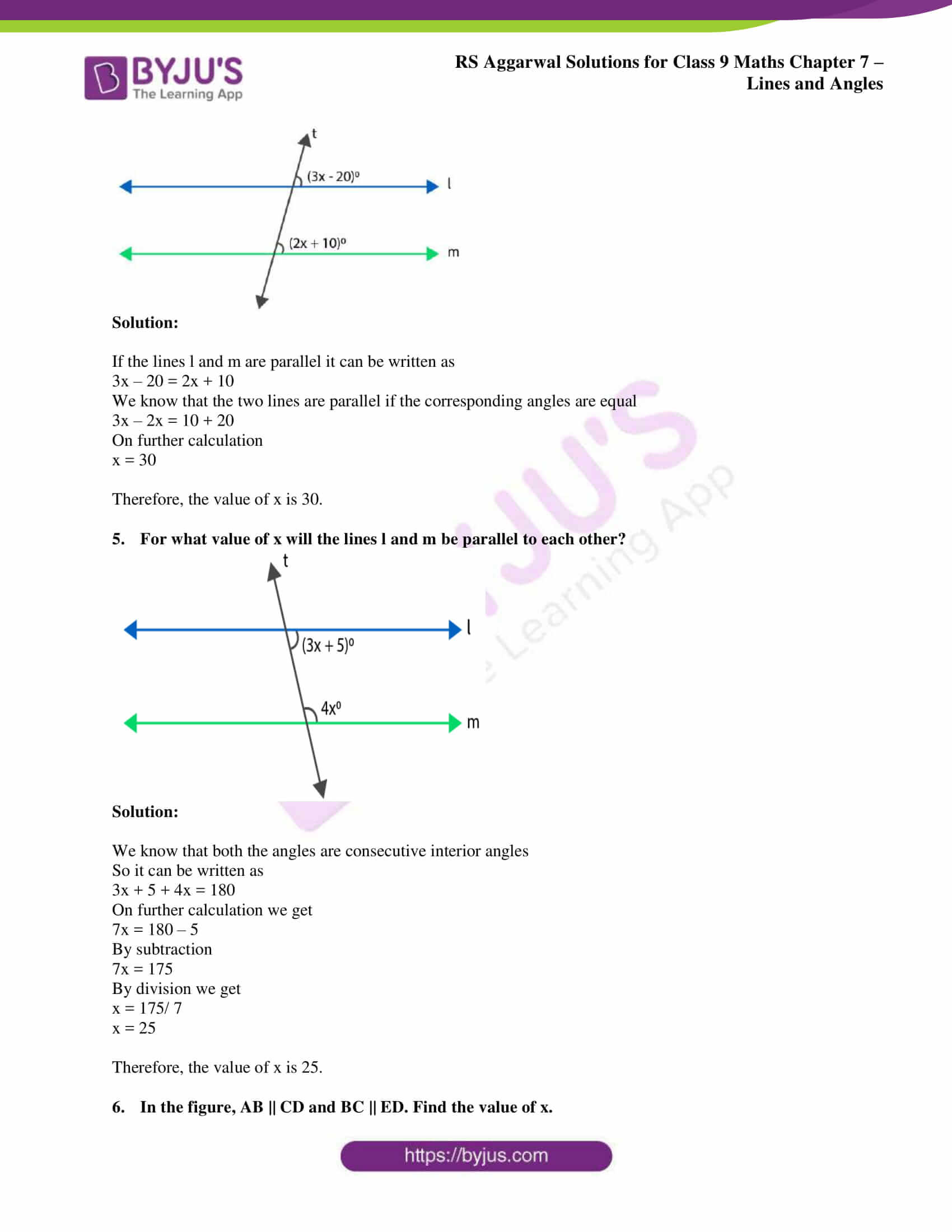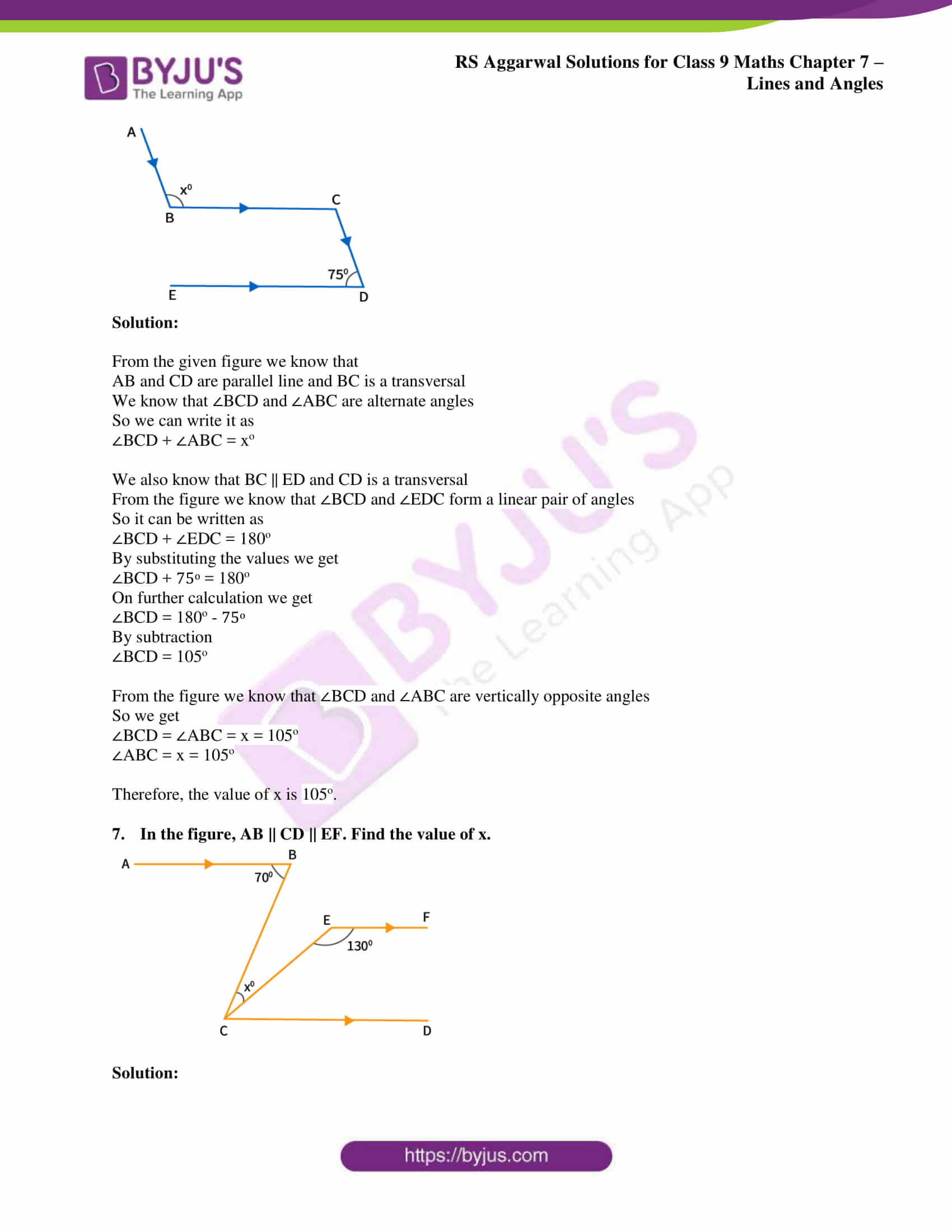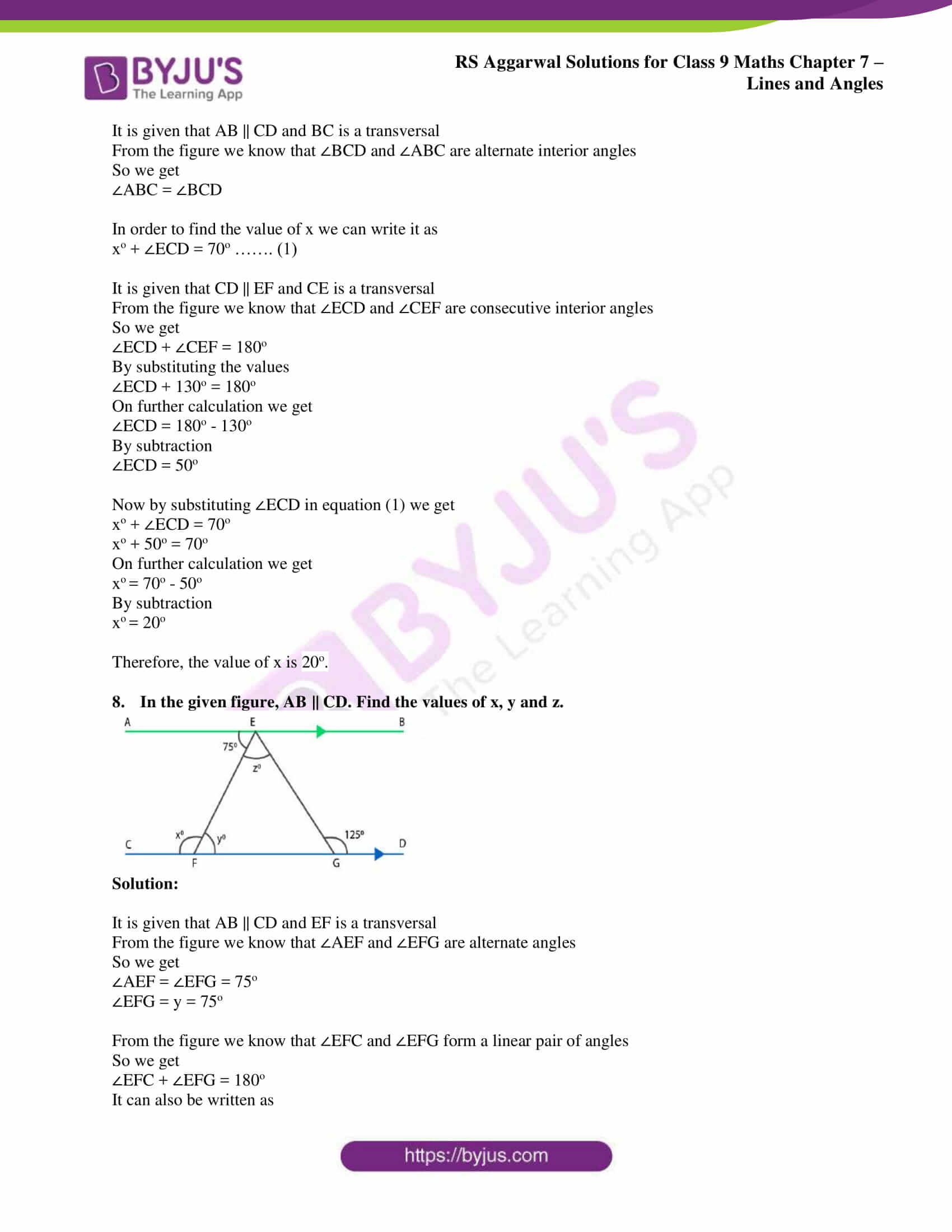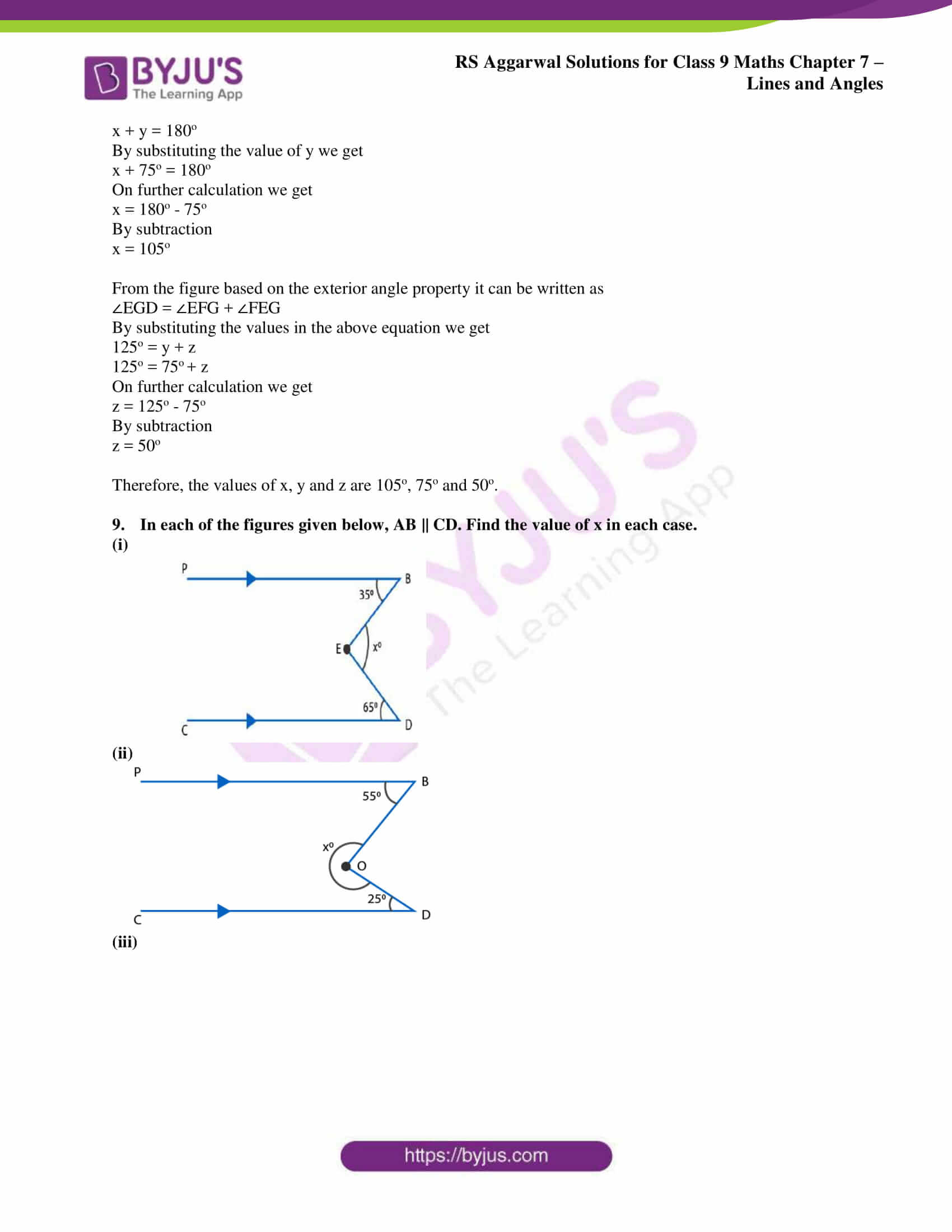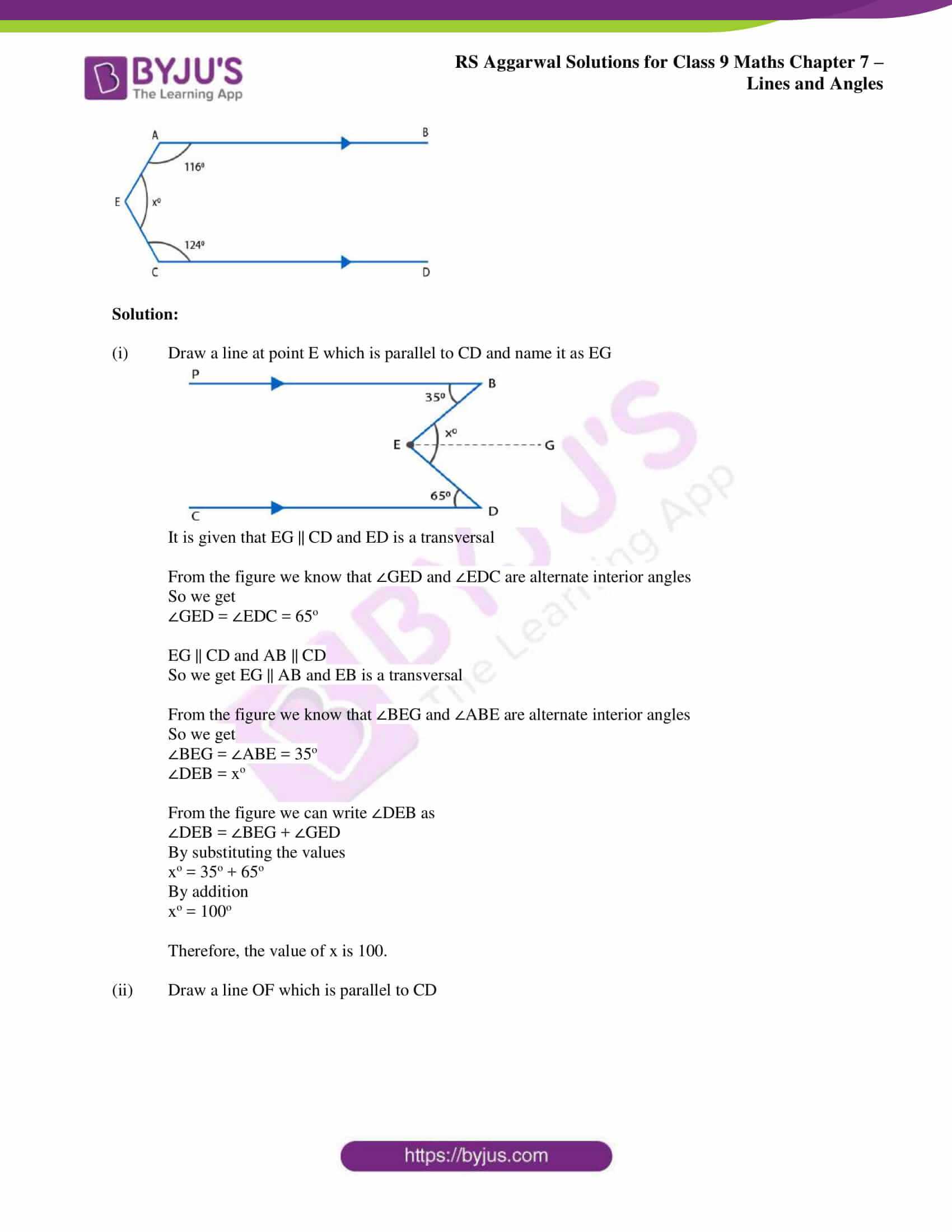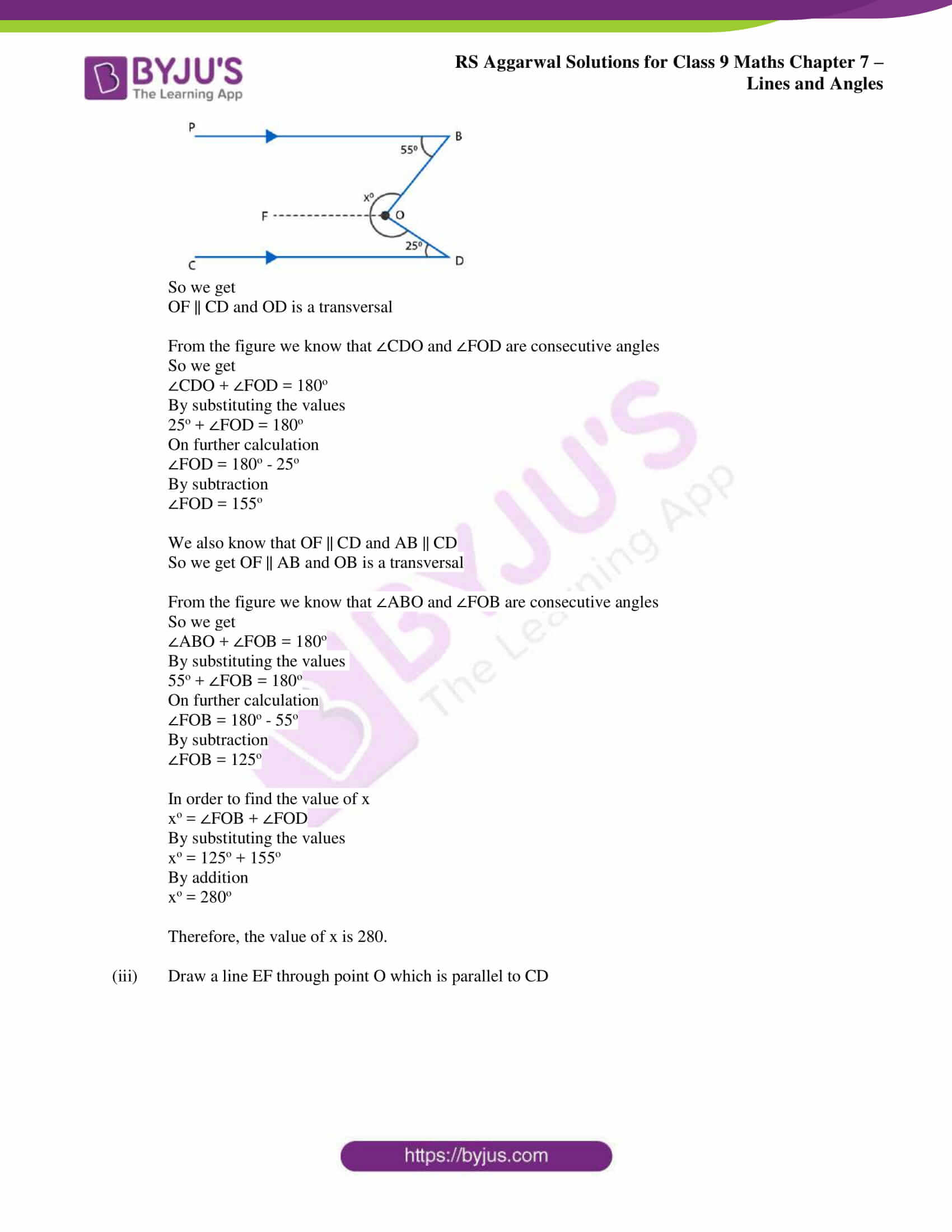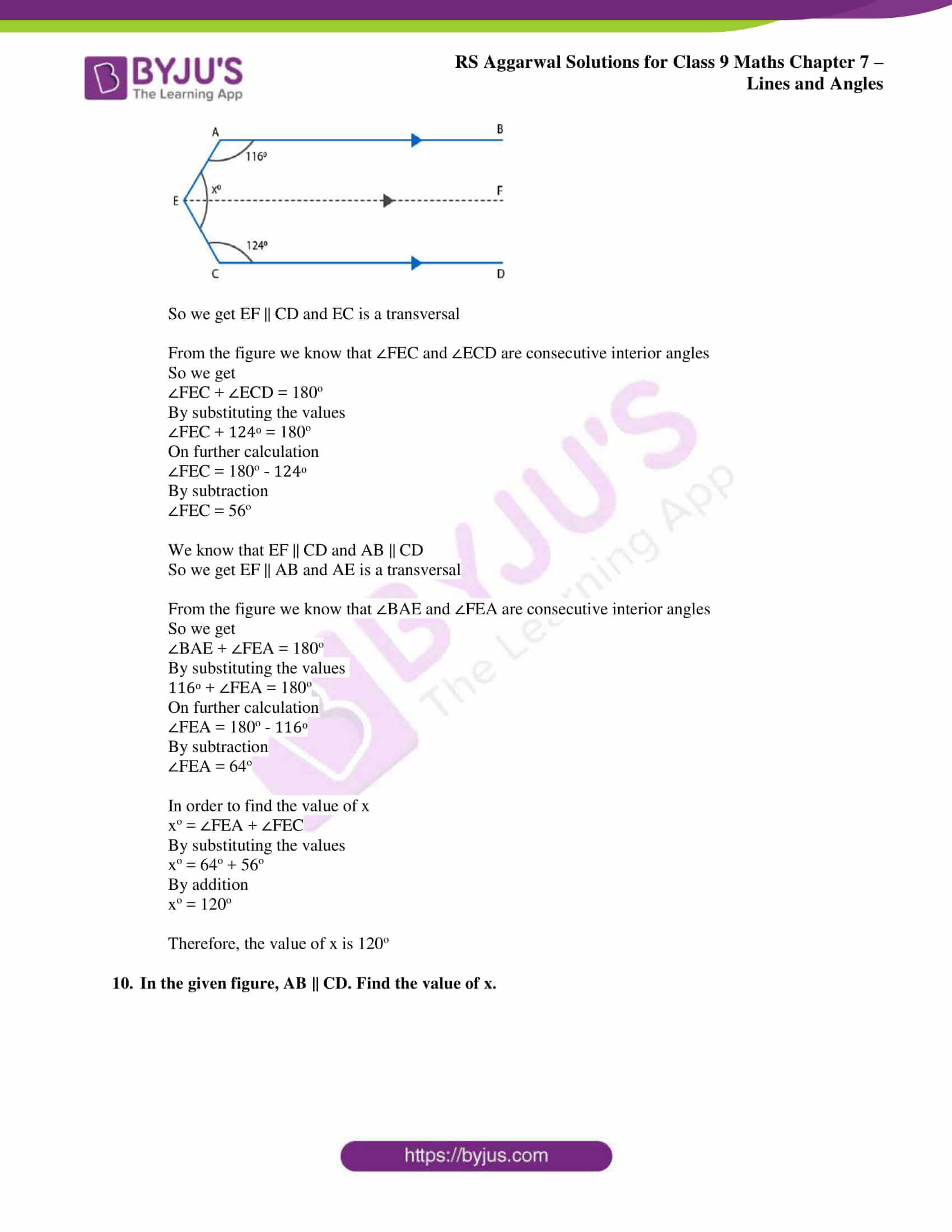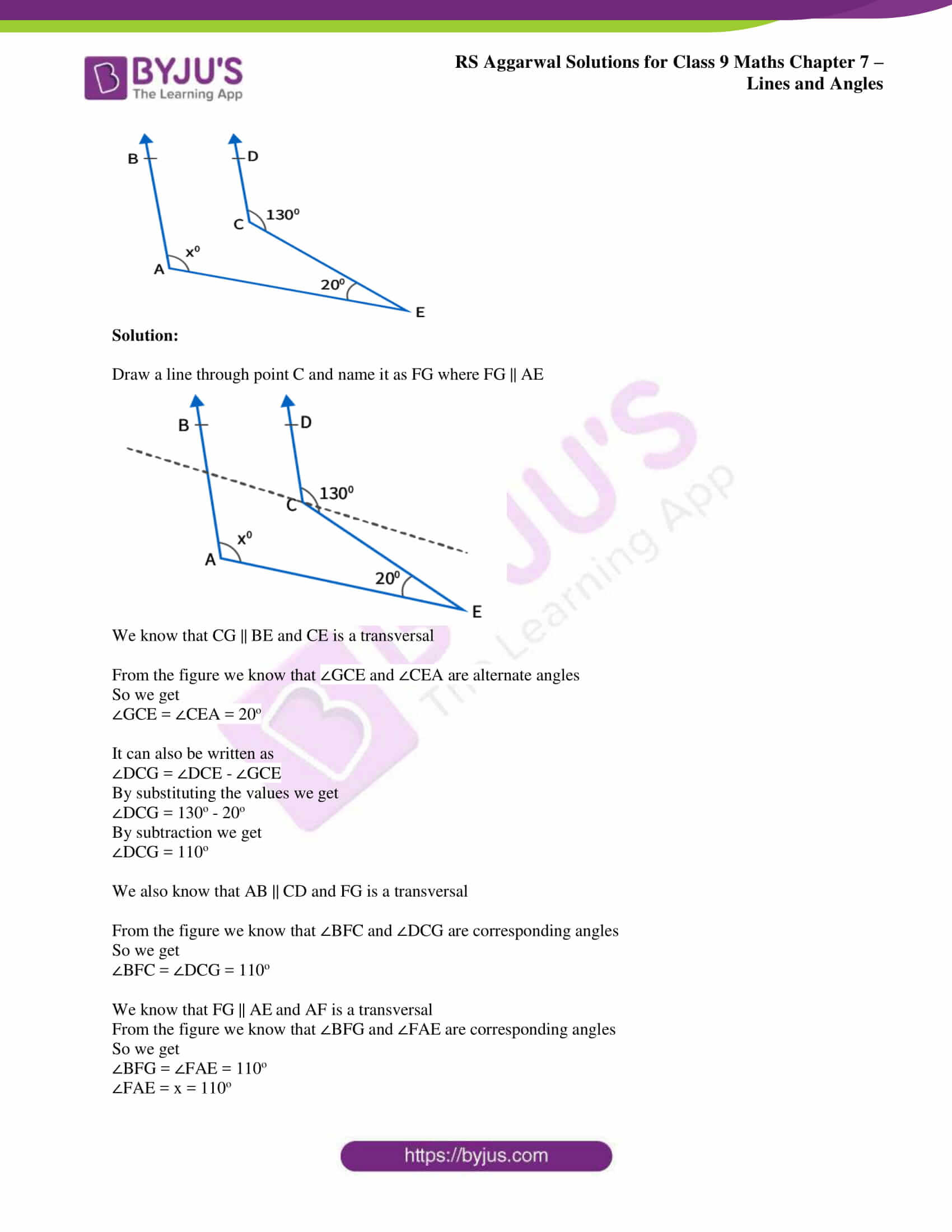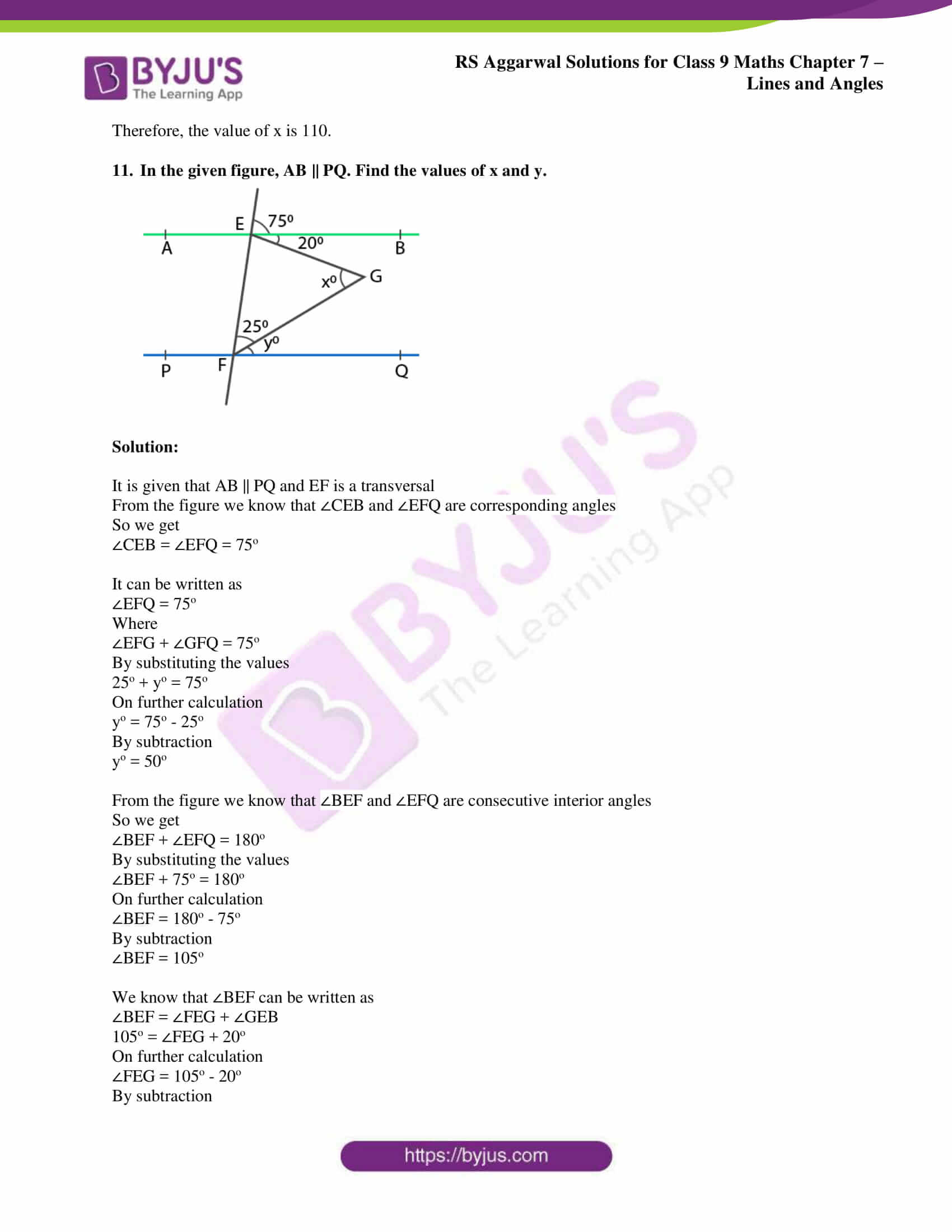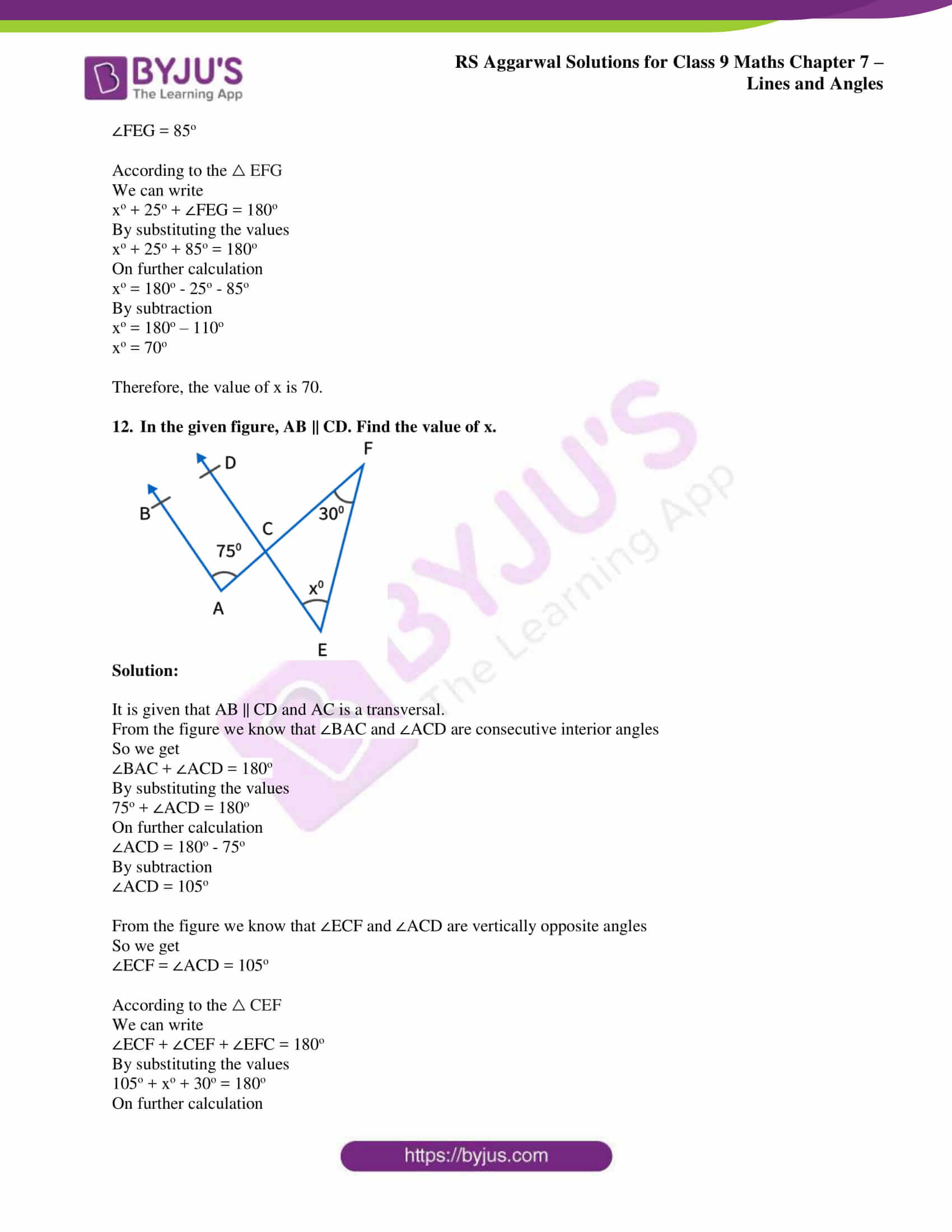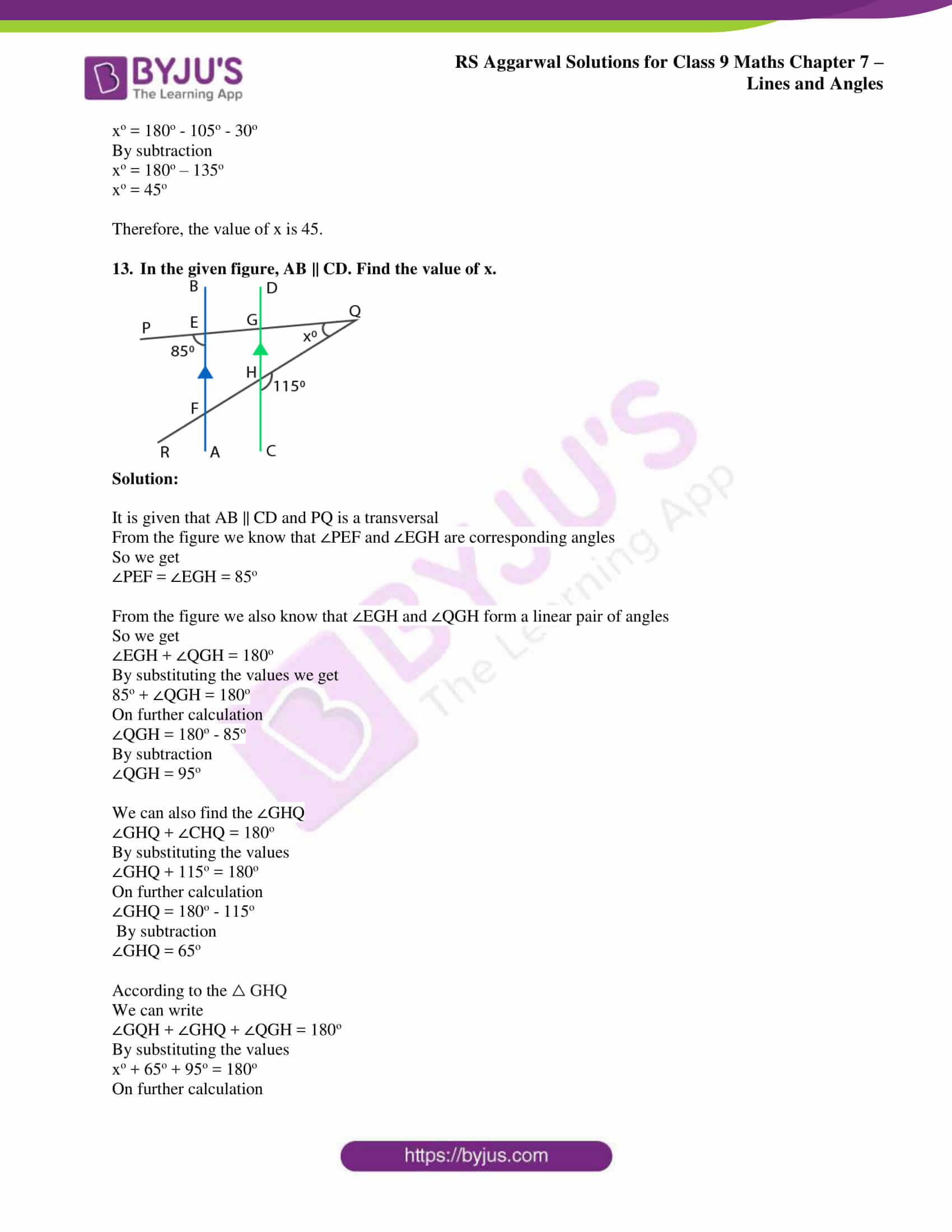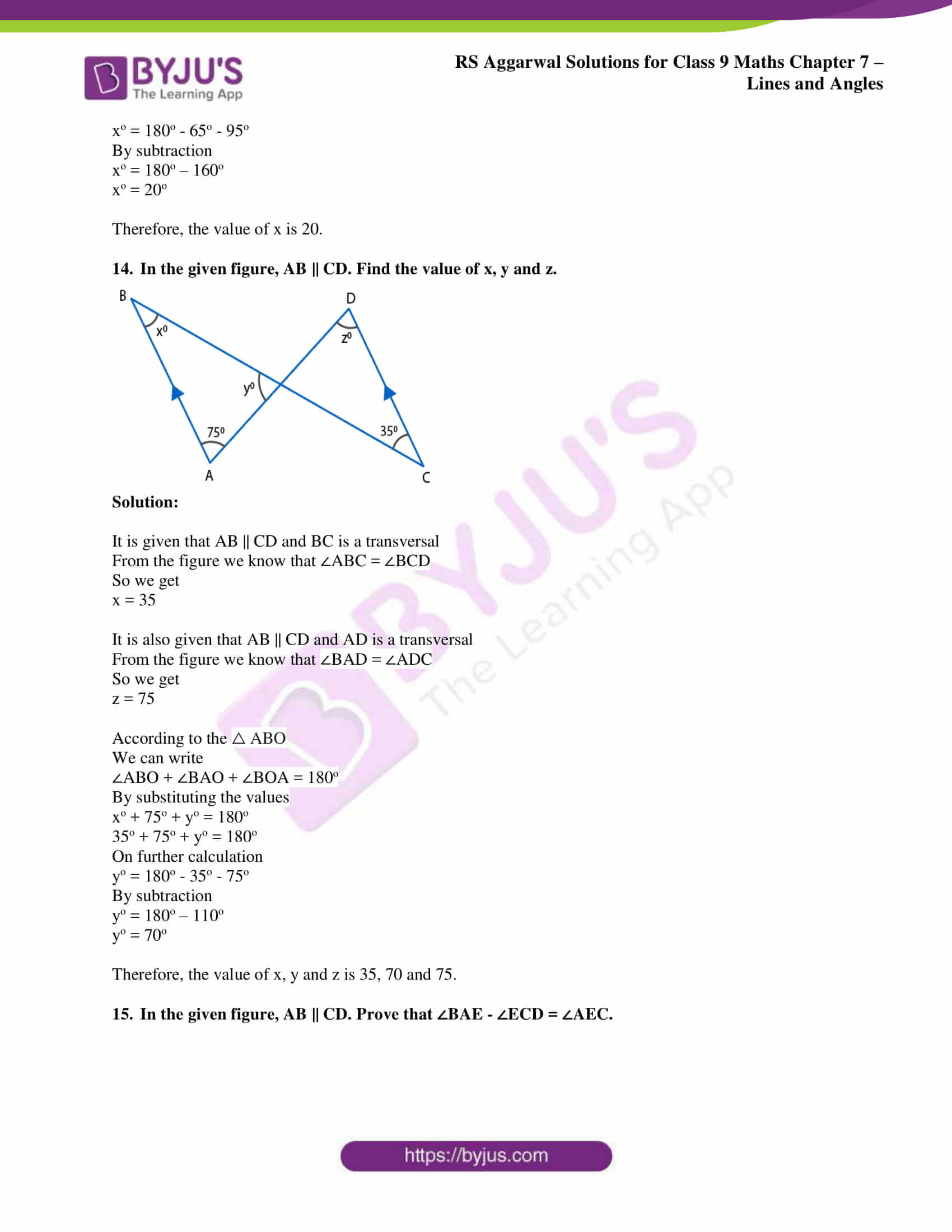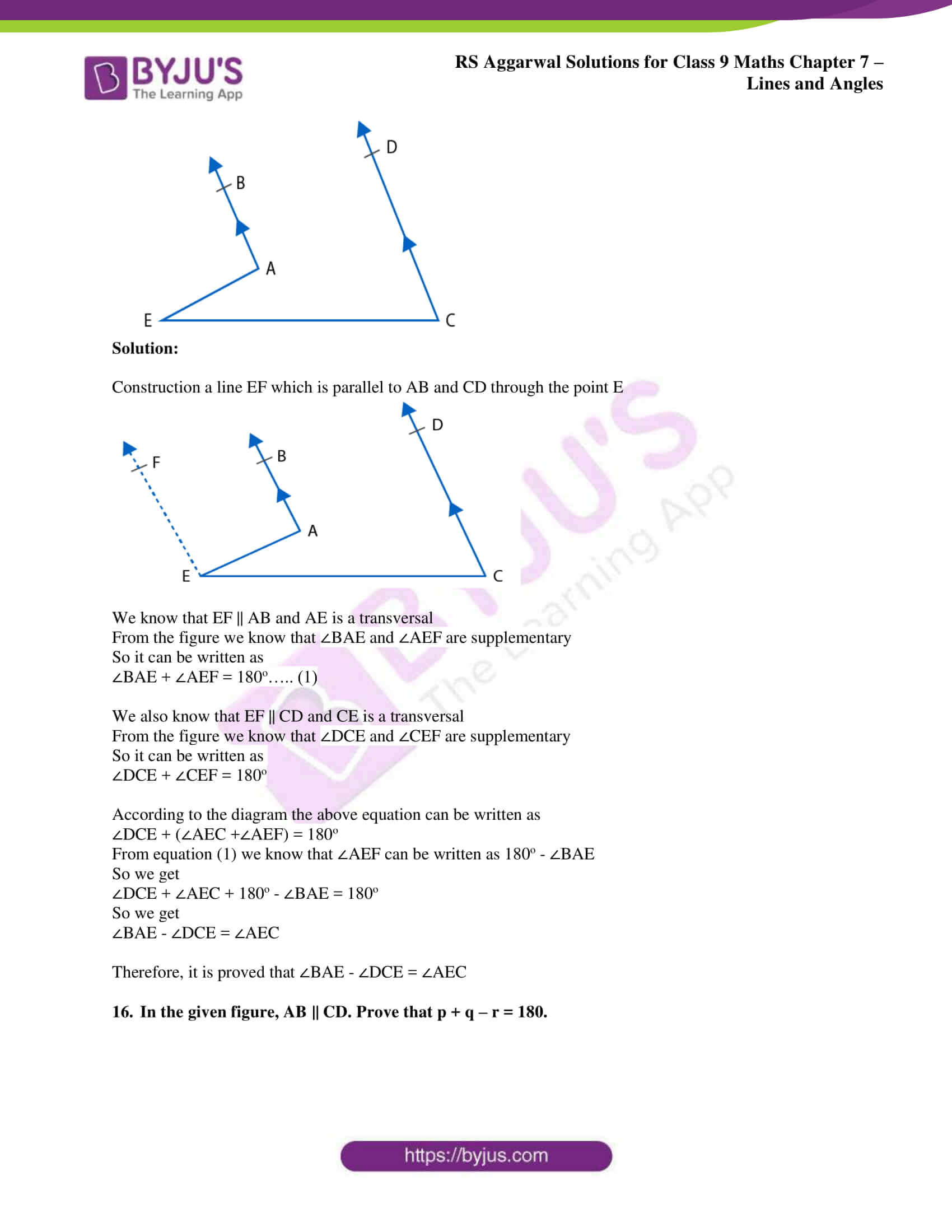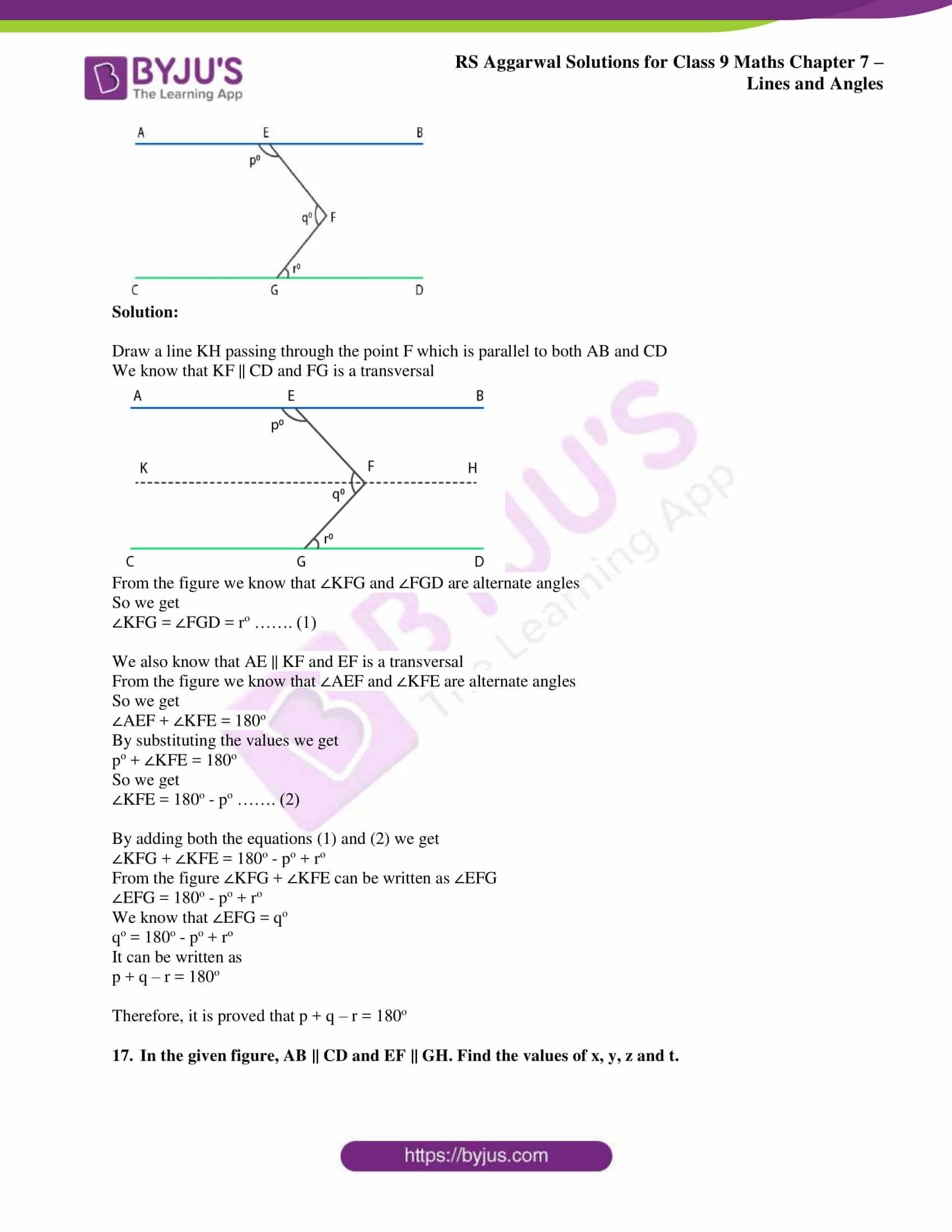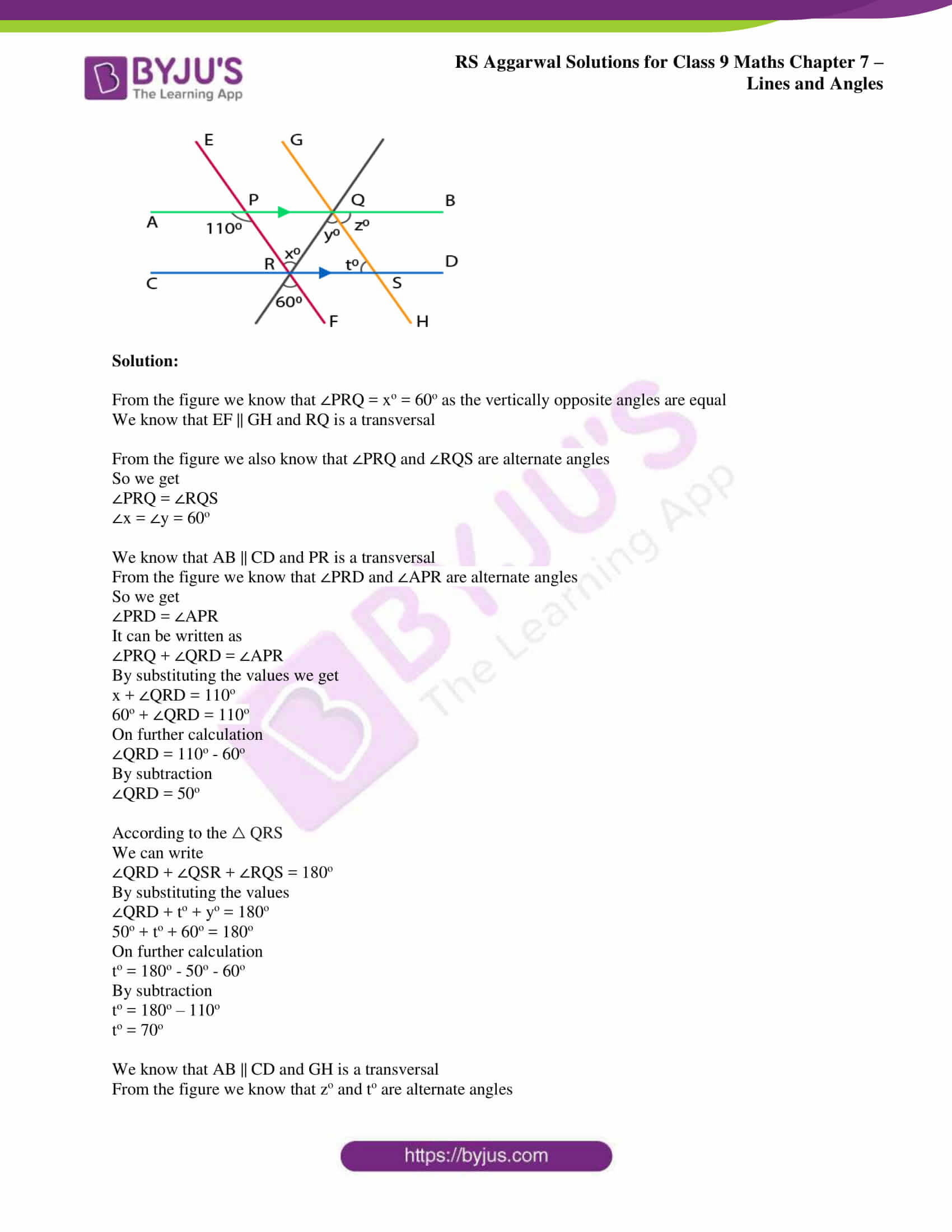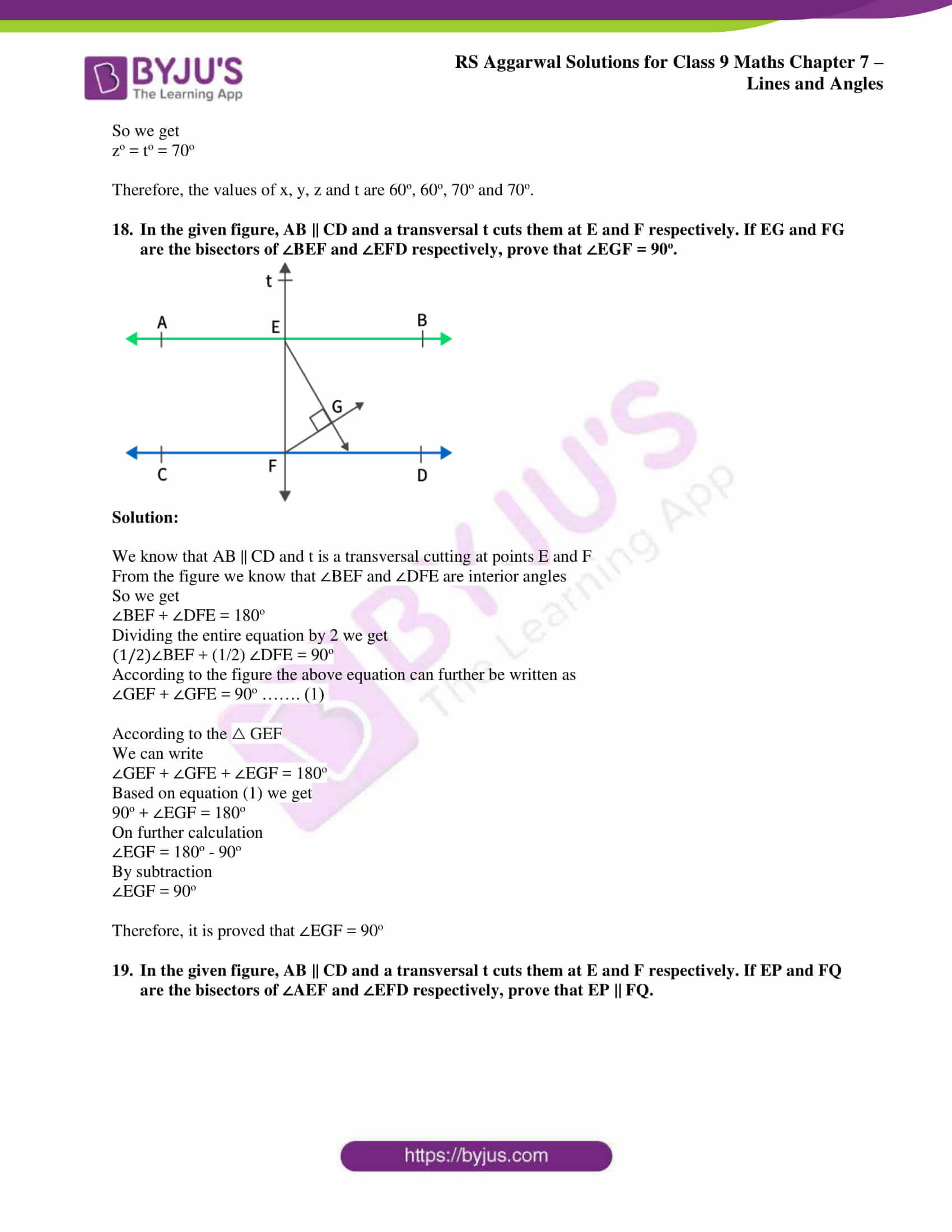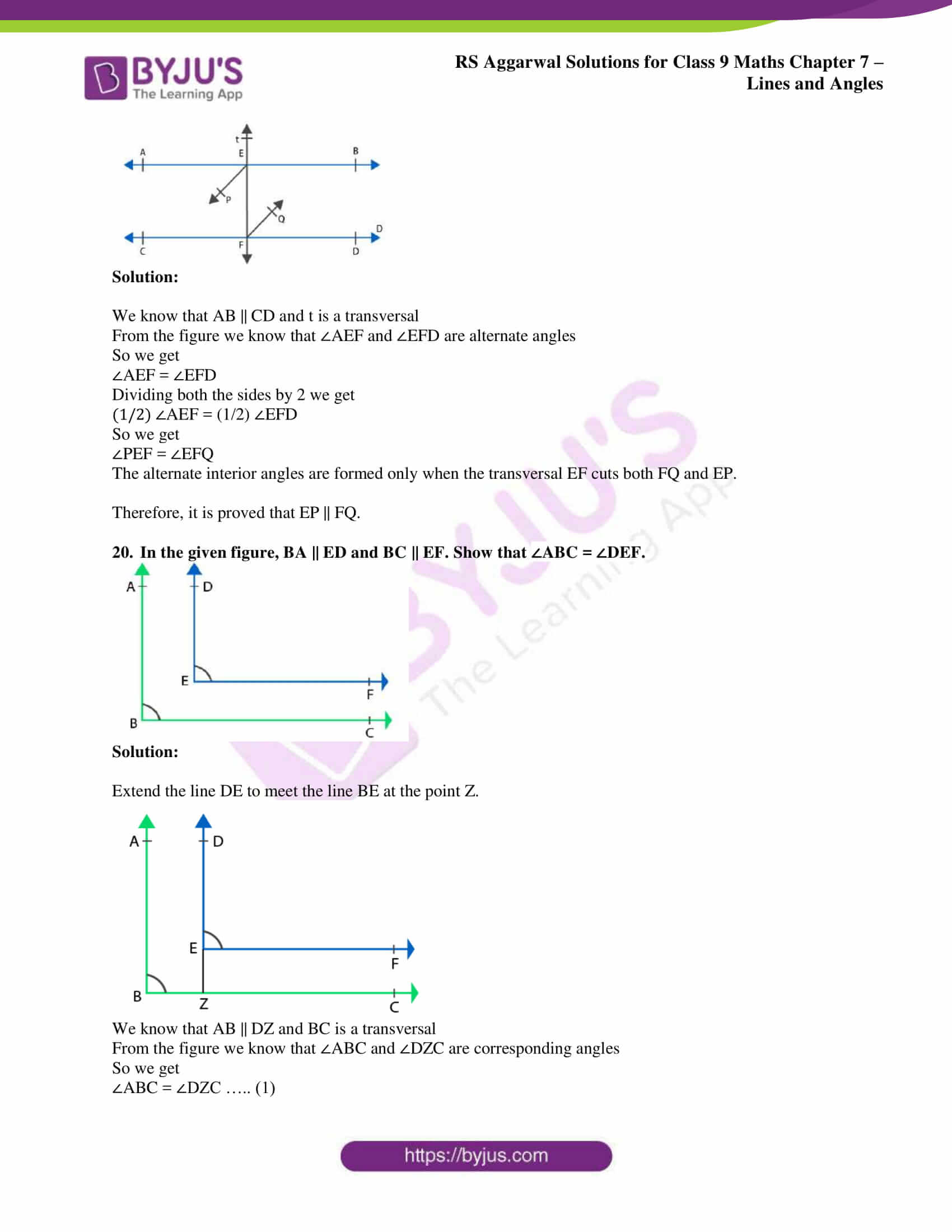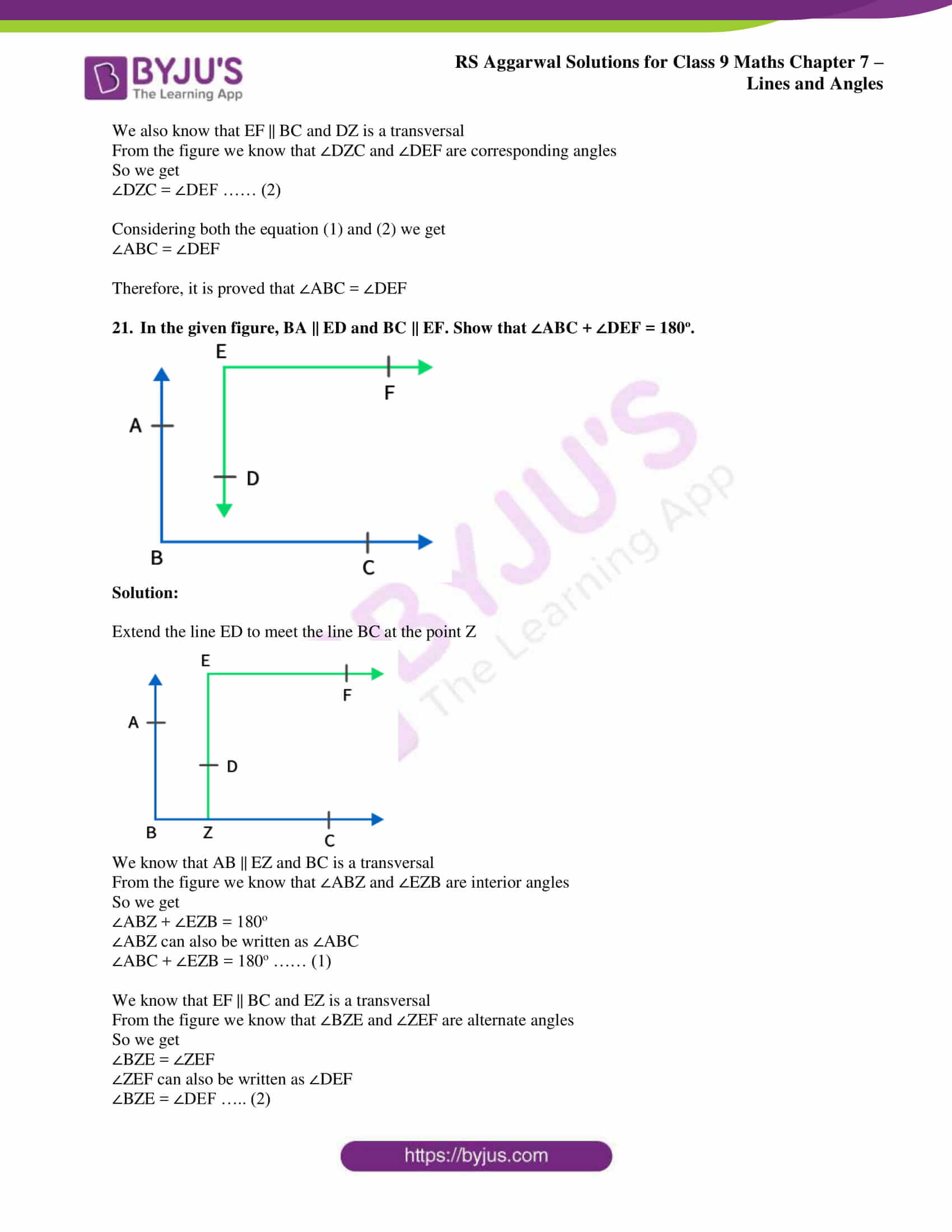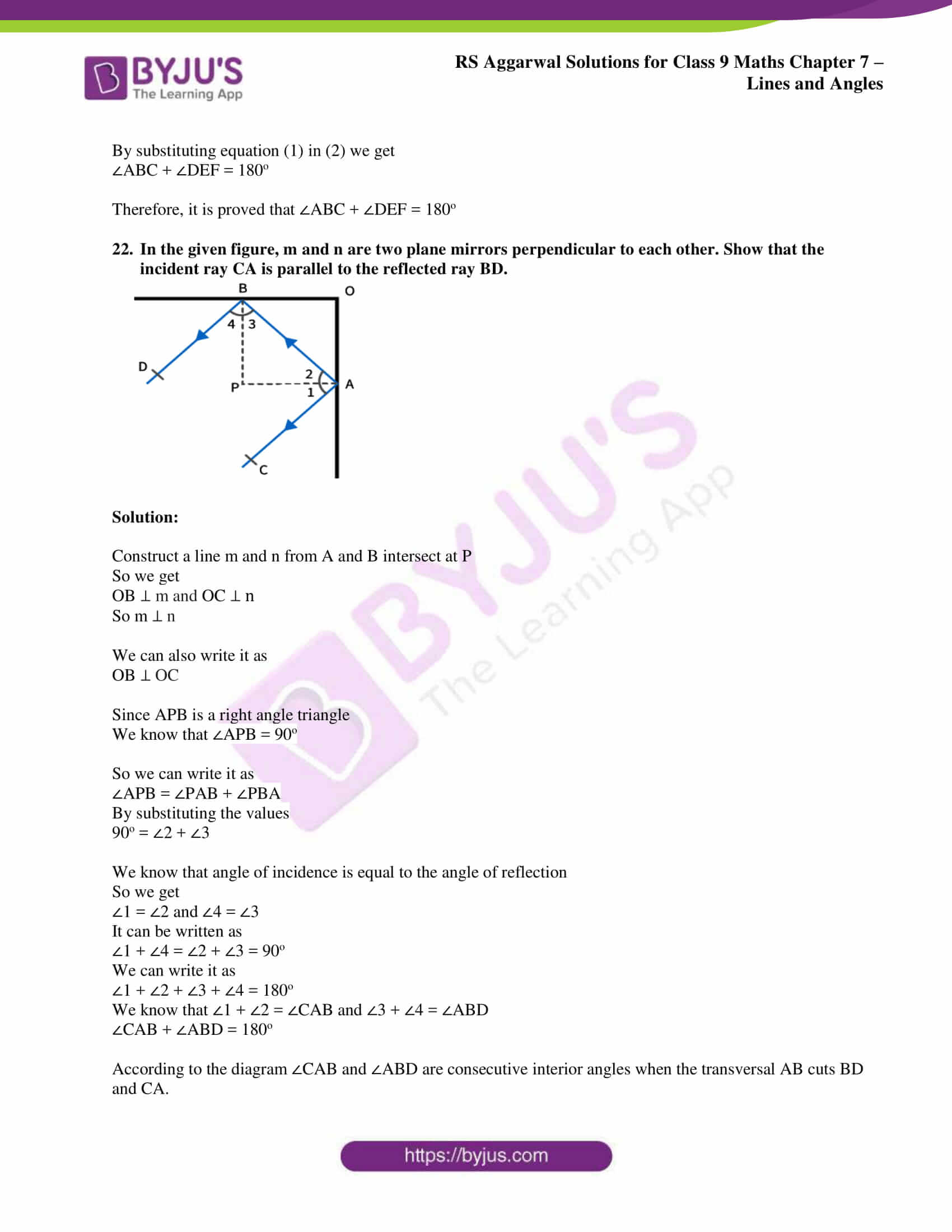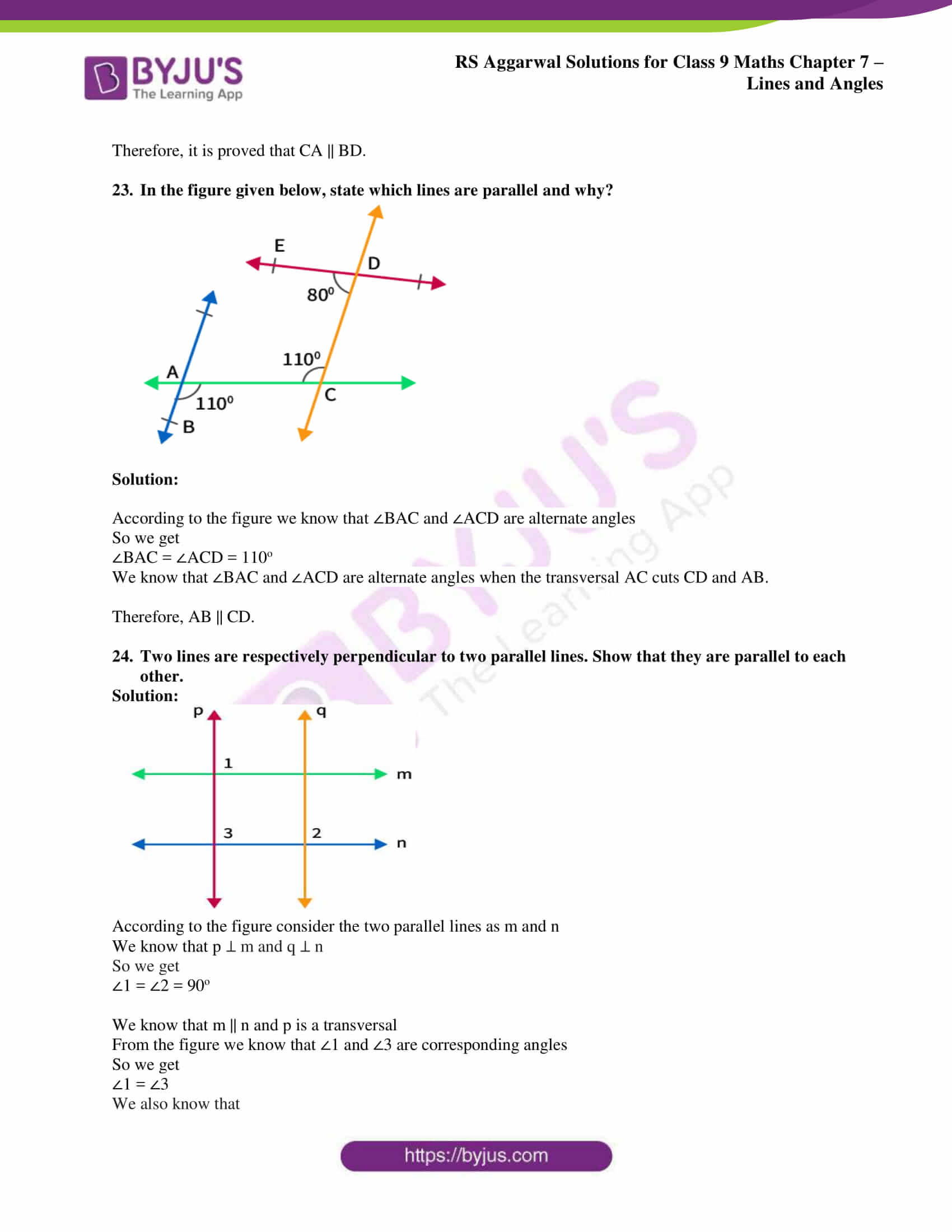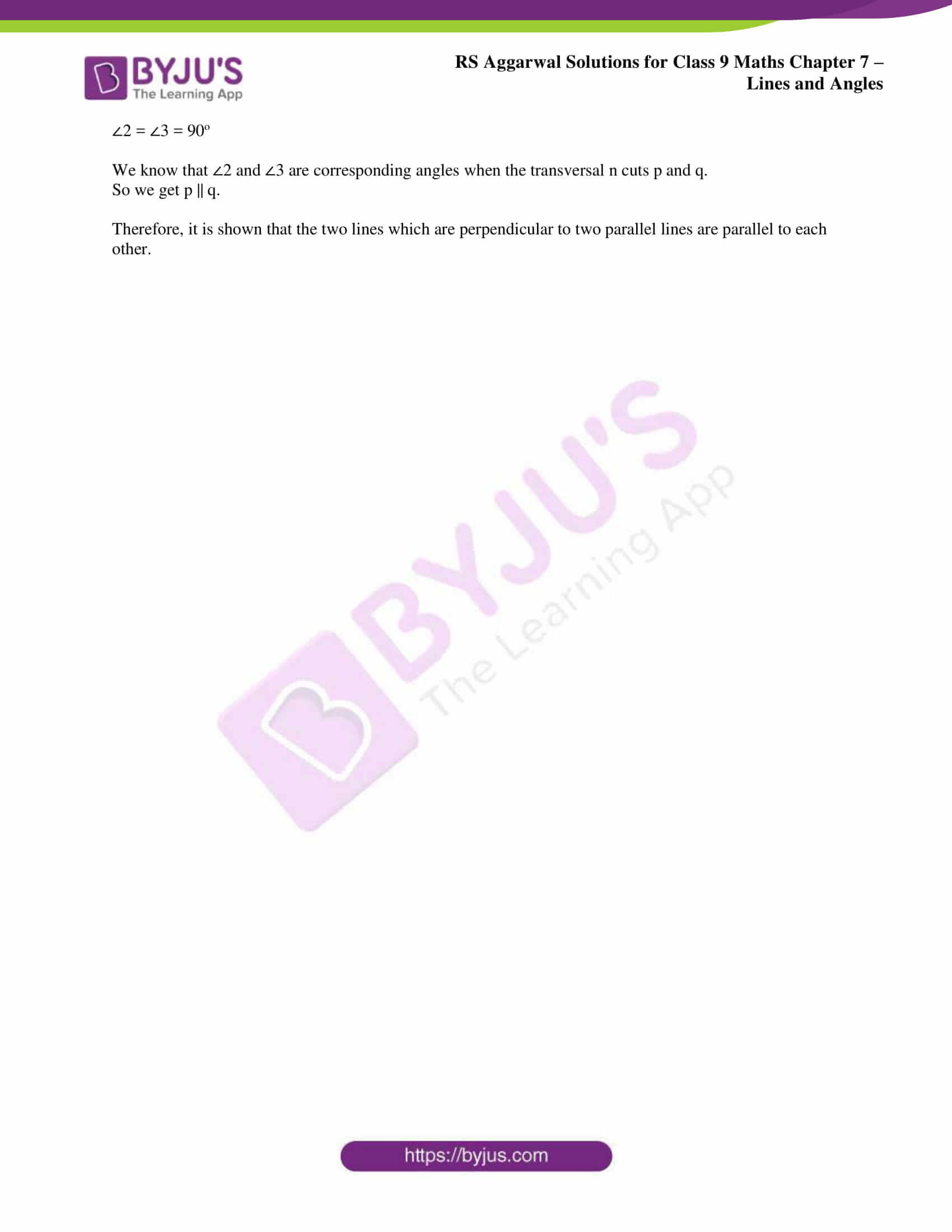## Access RS Aggarwal Solutions for Class 9 Chapter 7: Lines and Angles Exercise 7C

Exercise 7(C)

1. In the given figure, l || m and a transversal t cuts them. If ∠1 = 120o, find the measure of each of the remaining marked angles.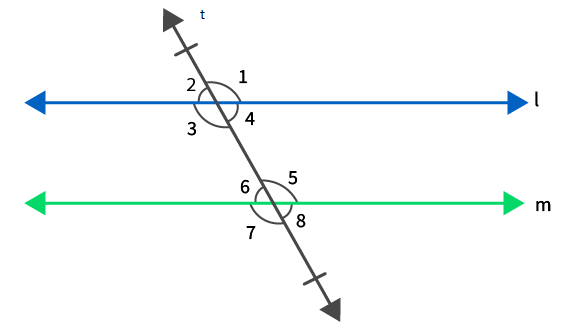Solution:

It is given that ∠1 = 120o

From the figure we know that ∠1 and ∠2 form a linear pair of angles

So it can be written as

∠1 + ∠2 = 180o

By substituting the values

120o + ∠2 = 180o

On further calculation

∠2 = 180o – 120o

By subtraction

∠2 = 60o

From the figure we know that ∠1 and ∠3 are vertically opposite angles

So we get

∠1 = ∠3 = 120o

From the figure we know that ∠2 and ∠4 are vertically opposite angles

So we get

∠2 = ∠4 = 60o

It is given that, l || m and t is a transversal

So the corresponding angles according to the figure is written as

∠1 = ∠5 = 120o

∠2 = ∠6 = 60o

∠3 = ∠7 = 120o

∠4 = ∠8 = 60o

2. In the figure, l || m and a transversal t cuts them. If ∠7 = 80o, find the measure of each of the remaining marked angles.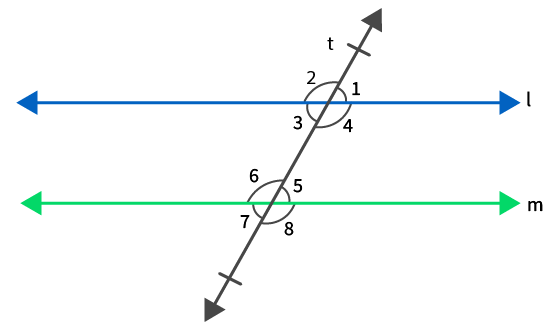Solution:

It is given that ∠7 = 80o

From the figure we know that ∠7 and ∠8 form a linear pair of angles

So it can be written as

∠7 + ∠8 = 180o

By substituting the values

80o + ∠8 = 180o

On further calculation

∠8 = 180o – 80o

By subtraction

∠8 = 100o

From the figure we know that ∠7 and ∠5 are vertically opposite angles

So we get

∠7 = ∠5 = 80o

From the figure we know that ∠6 and ∠8 are vertically opposite angles

So we get

∠6 = ∠8 = 100o

It is given that, l || m and t is a transversal

So the corresponding angles according to the figure is written as

∠1 = ∠5 = 80o

∠2 = ∠6 = 100o

∠3 = ∠7 = 80o

∠4 = ∠8 = 100o

3. In the figure, l || m and a transversal t cuts them. If ∠1: ∠2 = 2: 3, find the measure of each of the marked angles.Solution:

It is given that ∠1: ∠2 = 2: 3

From the figure we know that ∠1 and ∠2 form a linear pair of angles

So it can be written as

∠1 + ∠2 = 180o

By substituting the values

2x + 3x = 180o

On further calculation

5x = 180o

By division

x = 180o/5

x = 36o

By substituting the value of x we get

∠1 = 2x = 2 (36o) = 72o

∠2 = 3x = 3 (36o) = 108o

From the figure we know that ∠1 and ∠3 are vertically opposite angles

So we get

∠1 = ∠3 = 72o

From the figure we know that ∠2 and ∠4 are vertically opposite angles

So we get

∠2 = ∠4 = 108o

It is given that, l || m and t is a transversal

So the corresponding angles according to the figure is written as

∠1 = ∠5 = 72o

∠2 = ∠6 = 108o

∠3 = ∠7 = 72o

∠4 = ∠8 = 108o

4. For what value of x will the lines l and m be parallel to each other?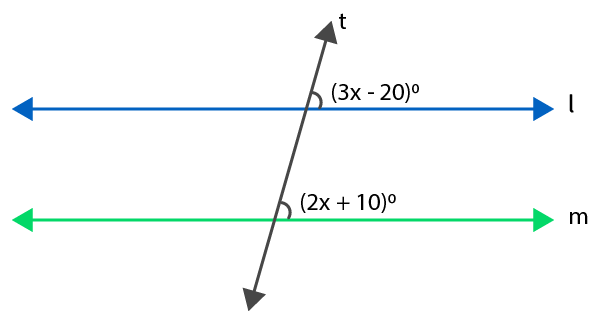Solution:

If the lines l and m are parallel it can be written as

3x – 20 = 2x + 10

We know that the two lines are parallel if the corresponding angles are equal

3x – 2x = 10 + 20

On further calculation

x = 30

Therefore, the value of x is 30.

5. For what value of x will the lines l and m be parallel to each other?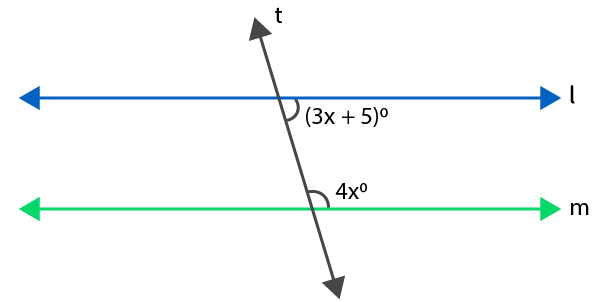Solution:

We know that both the angles are consecutive interior angles

So it can be written as

3x + 5 + 4x = 180

On further calculation we get

7x = 180 – 5

By subtraction

7x = 175

By division we get

x = 175/ 7

x = 25

Therefore, the value of x is 25.

6. In the figure, AB || CD and BC || ED. Find the value of x.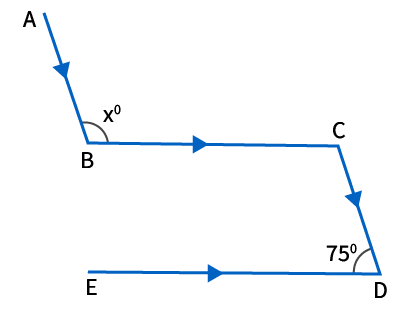Solution:

From the given figure we know that

AB and CD are parallel line and BC is a transversal

We know that ∠BCD and ∠ABC are alternate angles

So we can write it as

∠BCD + ∠ABC = xo

We also know that BC || ED and CD is a transversal

From the figure we know that ∠BCD and ∠EDC form a linear pair of angles

So it can be written as

∠BCD + ∠EDC = 180o

By substituting the values we get

∠BCD + 75o = 180o

On further calculation we get

∠BCD = 180o – 75o

By subtraction

∠BCD = 105o

From the figure we know that ∠BCD and ∠ABC are vertically opposite angles

So we get

∠BCD = ∠ABC = x = 105o

∠ABC = x = 105o

Therefore, the value of x is 105o.

7. In the figure, AB || CD || EF. Find the value of x.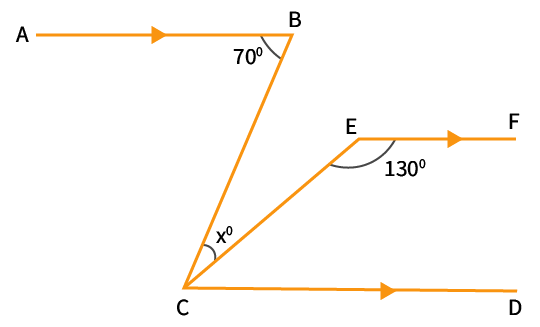Solution:

It is given that AB || CD and BC is a transversal

From the figure we know that ∠BCD and ∠ABC are alternate interior angles

So we get

∠ABC = ∠BCD

In order to find the value of x we can write it as

xo + ∠ECD = 70o ……. (1)

It is given that CD || EF and CE is a transversal

From the figure we know that ∠ECD and ∠CEF are consecutive interior angles

So we get

∠ECD + ∠CEF = 180o

By substituting the values

∠ECD + 130o = 180o

On further calculation we get

∠ECD = 180o – 130o

By subtraction

∠ECD = 50o

Now by substituting ∠ECD in equation (1) we get

xo + ∠ECD = 70o

xo + 50o = 70o

On further calculation we get

xo = 70o – 50o

By subtraction

xo = 20o

Therefore, the value of x is 20o.

8. In the given figure, AB || CD. Find the values of x, y and z.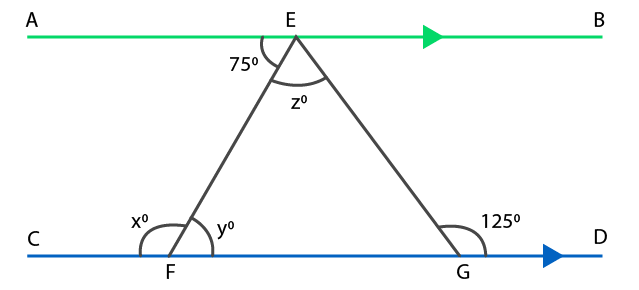Solution:

It is given that AB || CD and EF is a transversal

From the figure we know that ∠AEF and ∠EFG are alternate angles

So we get

∠AEF = ∠EFG = 75o

∠EFG = y = 75o

From the figure we know that ∠EFC and ∠EFG form a linear pair of angles

So we get

∠EFC + ∠EFG = 180o

It can also be written as

x + y = 180o

By substituting the value of y we get

x + 75o = 180o

On further calculation we get

x = 180o – 75o

By subtraction

x = 105o

From the figure based on the exterior angle property it can be written as

∠EGD = ∠EFG + ∠FEG

By substituting the values in the above equation we get

125o = y + z

125o = 75o + z

On further calculation we get

z = 125o – 75o

By subtraction

z = 50o

Therefore, the values of x, y and z are 105o, 75o and 50o.

9. In each of the figures given below, AB || CD. Find the value of x in each case.

(i)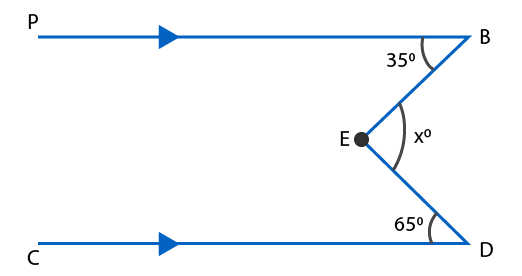(ii)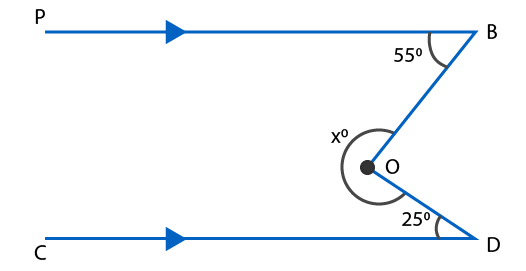(iii)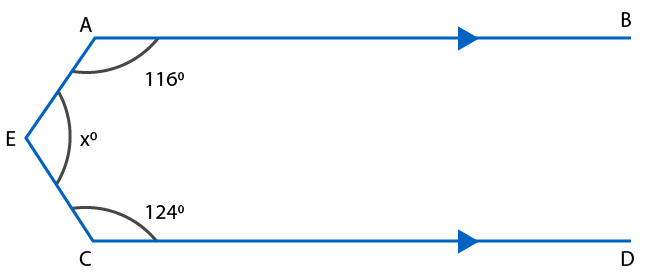Solution:

(i) Draw a line at point E which is parallel to CD and name it as EG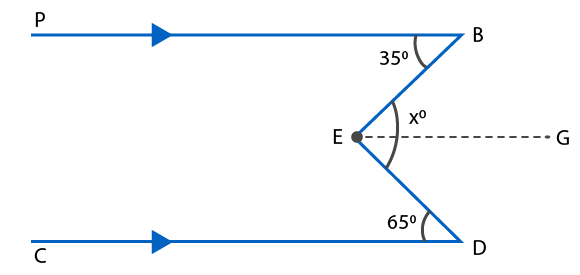It is given that EG || CD and ED is a transversal

From the figure we know that ∠GED and ∠EDC are alternate interior angles

So we get

∠GED = ∠EDC = 65o

EG || CD and AB || CD

So we get EG || AB and EB is a transversal

From the figure we know that ∠BEG and ∠ABE are alternate interior angles

So we get

∠BEG = ∠ABE = 35o

∠DEB = xo

From the figure we can write ∠DEB as

∠DEB = ∠BEG + ∠GED

By substituting the values

xo = 35o + 65o

xo = 100o

Therefore, the value of x is 100.

(ii) Draw a line OF which is parallel to CD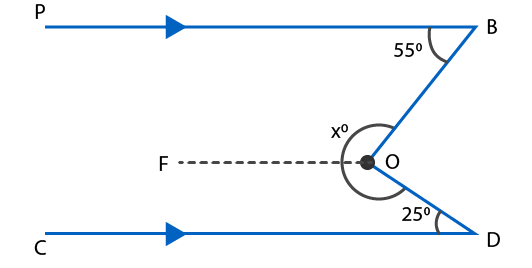So we get

OF || CD and OD is a transversal

From the figure we know that ∠CDO and ∠FOD are consecutive angles

So we get

∠CDO + ∠FOD = 180o

By substituting the values

25o + ∠FOD = 180o

On further calculation

∠FOD = 180o – 25o

By subtraction

∠FOD = 155o

We also know that OF || CD and AB || CD

So we get OF || AB and OB is a transversal

From the figure we know that ∠ABO and ∠FOB are consecutive angles

So we get

∠ABO + ∠FOB = 180o

By substituting the values

55o + ∠FOB = 180o

On further calculation

∠FOB = 180o – 55o

By subtraction

∠FOB = 125o

In order to find the value of x

xo = ∠FOB + ∠FOD

By substituting the values

xo = 125o + 155o

xo = 280o

Therefore, the value of x is 280.

(iii) Draw a line EF through point O which is parallel to CD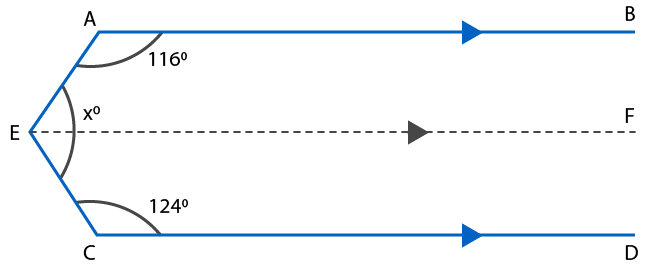So we get EF || CD and EC is a transversal

From the figure we know that ∠FEC and ∠ECD are consecutive interior angles

So we get

∠FEC + ∠ECD = 180o

By substituting the values

∠FEC + 124o = 180o

On further calculation

∠FEC = 180o – 124o

By subtraction

∠FEC = 56o

We know that EF || CD and AB || CD

So we get EF || AB and AE is a transversal

From the figure we know that ∠BAE and ∠FEA are consecutive interior angles

So we get

∠BAE + ∠FEA = 180o

By substituting the values

116o + ∠FEA = 180o

On further calculation

∠FEA = 180o – 116o

By subtraction

∠FEA = 64o

In order to find the value of x

xo = ∠FEA + ∠FEC

By substituting the values

xo = 64o + 56o

xo = 120o

Therefore, the value of x is 120o

10. In the given figure, AB || CD. Find the value of x.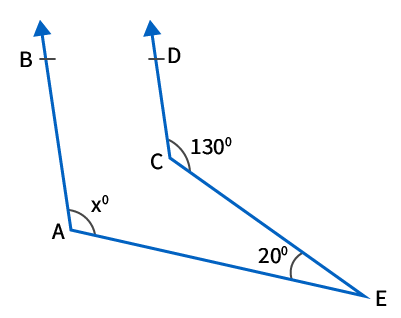Solution:

Draw a line through point C and name it as FG where FG || AE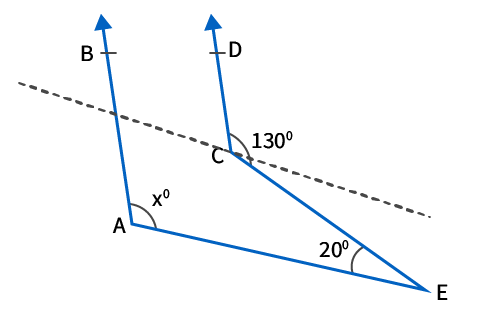We know that CG || BE and CE is a transversal

From the figure we know that ∠GCE and ∠CEA are alternate angles

So we get

∠GCE = ∠CEA = 20o

It can also be written as

∠DCG = ∠DCE – ∠GCE

By substituting the values we get

∠DCG = 130o – 20o

By subtraction we get

∠DCG = 110o

We also know that AB || CD and FG is a transversal

From the figure we know that ∠BFC and ∠DCG are corresponding angles

So we get

∠BFC = ∠DCG = 110o

We know that FG || AE and AF is a transversal

From the figure we know that ∠BFG and ∠FAE are corresponding angles

So we get

∠BFG = ∠FAE = 110o

∠FAE = x = 110o

Therefore, the value of x is 110.

11. In the given figure, AB || PQ. Find the values of x and y.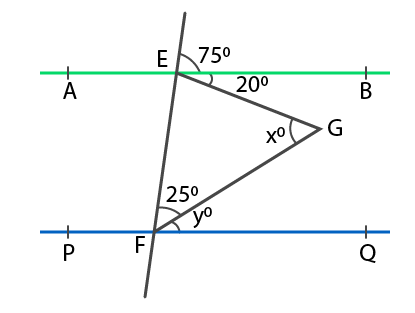Solution:

It is given that AB || PQ and EF is a transversal

From the figure we know that ∠CEB and ∠EFQ are corresponding angles

So we get

∠CEB = ∠EFQ = 75o

It can be written as

∠EFQ = 75o

Where

∠EFG + ∠GFQ = 75o

By substituting the values

25o + yo = 75o

On further calculation

yo = 75o – 25o

By subtraction

yo = 50o

From the figure we know that ∠BEF and ∠EFQ are consecutive interior angles

So we get

∠BEF + ∠EFQ = 180o

By substituting the values

∠BEF + 75o = 180o

On further calculation

∠BEF = 180o – 75o

By subtraction

∠BEF = 105o

We know that ∠BEF can be written as

∠BEF = ∠FEG + ∠GEB

105o = ∠FEG + 20o

On further calculation

∠FEG = 105o – 20o

By subtraction

∠FEG = 85o

According to the △ EFG

We can write

xo + 25o + ∠FEG = 180o

By substituting the values

xo + 25o + 85o = 180o

On further calculation

xo = 180o – 25o – 85o

By subtraction

xo = 180o – 110o

xo = 70o

Therefore, the value of x is 70.

12. In the given figure, AB || CD. Find the value of x.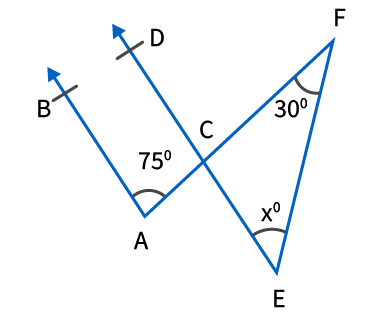Solution:

It is given that AB || CD and AC is a transversal.

From the figure we know that ∠BAC and ∠ACD are consecutive interior angles

So we get

∠BAC + ∠ACD = 180o

By substituting the values

75o + ∠ACD = 180o

On further calculation

∠ACD = 180o – 75o

By subtraction

∠ACD = 105o

From the figure we know that ∠ECF and ∠ACD are vertically opposite angles

So we get

∠ECF = ∠ACD = 105o

According to the △ CEF

We can write

∠ECF + ∠CEF + ∠EFC = 180o

By substituting the values

105o + xo + 30o = 180o

On further calculation

xo = 180o – 105o – 30o

By subtraction

xo = 180o – 135o

xo = 45o

Therefore, the value of x is 45.

13. In the given figure, AB || CD. Find the value of x.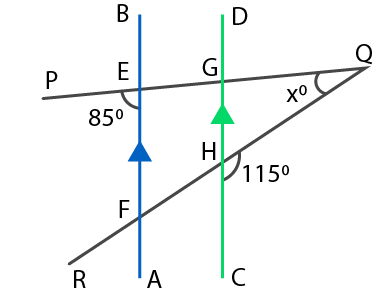Solution:

It is given that AB || CD and PQ is a transversal

From the figure we know that ∠PEF and ∠EGH are corresponding angles

So we get

∠PEF = ∠EGH = 85o

From the figure we also know that ∠EGH and ∠QGH form a linear pair of angles

So we get

∠EGH + ∠QGH = 180o

By substituting the values we get

85o + ∠QGH = 180o

On further calculation

∠QGH = 180o – 85o

By subtraction

∠QGH = 95o

We can also find the ∠GHQ

∠GHQ + ∠CHQ = 180o

By substituting the values

∠GHQ + 115o = 180o

On further calculation

∠GHQ = 180o – 115o

By subtraction

∠GHQ = 65o

According to the △ GHQ

We can write

∠GQH + ∠GHQ + ∠QGH = 180o

By substituting the values

xo + 65o + 95o = 180o

On further calculation

xo = 180o – 65o – 95o

By subtraction

xo = 180o – 160o

xo = 20o

Therefore, the value of x is 20.

14. In the given figure, AB || CD. Find the value of x, y and z.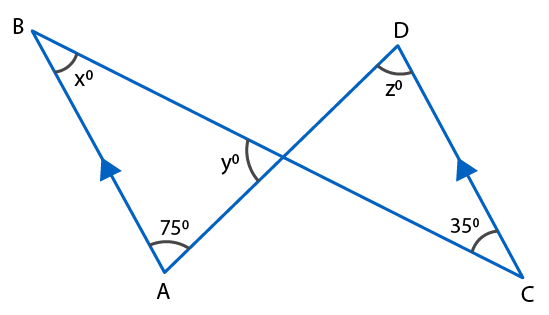Solution:

It is given that AB || CD and BC is a transversal

From the figure we know that ∠ABC = ∠BCD

So we get

x = 35

It is also given that AB || CD and AD is a transversal

So we get

z = 75

According to the △ ABO

We can write

∠ABO + ∠BAO + ∠BOA = 180o

By substituting the values

xo + 75o + yo = 180o

35o + 75o + yo = 180o

On further calculation

yo = 180o – 35o – 75o

By subtraction

yo = 180o – 110o

yo = 70o

Therefore, the value of x, y and z is 35, 70 and 75.

15. In the given figure, AB || CD. Prove that ∠BAE – ∠ECD = ∠AEC.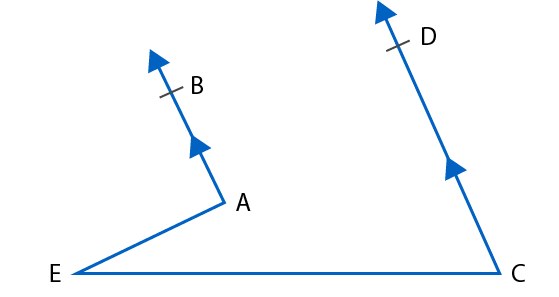Solution:

Construction a line EF which is parallel to AB and CD through the point E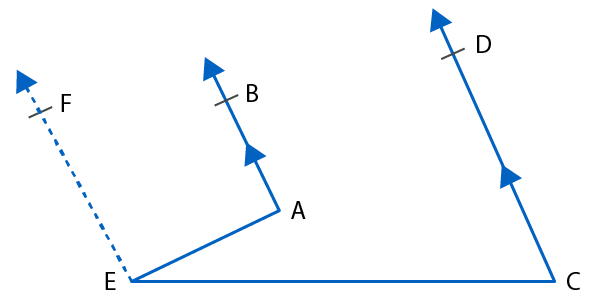We know that EF || AB and AE is a transversal

From the figure we know that ∠BAE and ∠AEF are supplementary

So it can be written as

∠BAE + ∠AEF = 180o….. (1)

We also know that EF || CD and CE is a transversal

From the figure we know that ∠DCE and ∠CEF are supplementary

So it can be written as

∠DCE + ∠CEF = 180o

According to the diagram the above equation can be written as

∠DCE + (∠AEC +∠AEF) = 180o

From equation (1) we know that ∠AEF can be written as 180o – ∠BAE

So we get

∠DCE + ∠AEC + 180o – ∠BAE = 180o

So we get

∠BAE – ∠DCE = ∠AEC

Therefore, it is proved that ∠BAE – ∠DCE = ∠AEC

16. In the given figure, AB || CD. Prove that p + q – r = 180.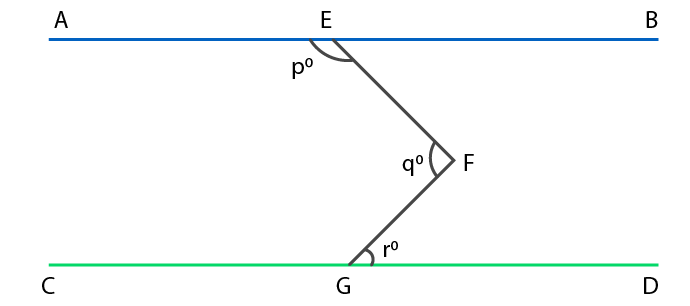Solution:

Draw a line KH passing through the point F which is parallel to both AB and CD

We know that KF || CD and FG is a transversal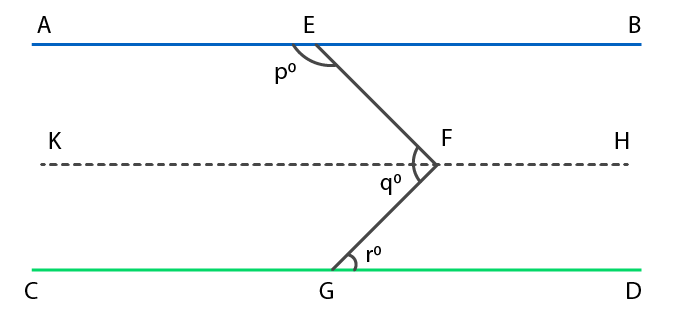From the figure we know that ∠KFG and ∠FGD are alternate angles

So we get

∠KFG = ∠FGD = ro ……. (1)

We also know that AE || KF and EF is a transversal

From the figure we know that ∠AEF and ∠KFE are alternate angles

So we get

∠AEF + ∠KFE = 180o

By substituting the values we get

po + ∠KFE = 180o

So we get

∠KFE = 180o – po ……. (2)

By adding both the equations (1) and (2) we get

∠KFG + ∠KFE = 180o – po + ro

From the figure ∠KFG + ∠KFE can be written as ∠EFG

∠EFG = 180o – po + ro

We know that ∠EFG = qo

qo = 180o – po + ro

It can be written as

p + q – r = 180o

Therefore, it is proved that p + q – r = 180o

17. In the given figure, AB || CD and EF || GH. Find the values of x, y, z and t.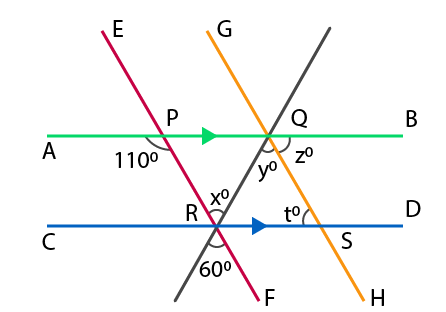Solution:

From the figure we know that ∠PRQ = xo = 60o as the vertically opposite angles are equal

We know that EF || GH and RQ is a transversal

From the figure we also know that ∠PRQ and ∠RQS are alternate angles

So we get

∠PRQ = ∠RQS

∠x = ∠y = 60o

We know that AB || CD and PR is a transversal

From the figure we know that ∠PRD and ∠APR are alternate angles

So we get

∠PRD = ∠APR

It can be written as

∠PRQ + ∠QRD = ∠APR

By substituting the values we get

x + ∠QRD = 110o

60o + ∠QRD = 110o

On further calculation

∠QRD = 110o – 60o

By subtraction

∠QRD = 50o

According to the △ QRS

We can write

∠QRD + ∠QSR + ∠RQS = 180o

By substituting the values

∠QRD + to + yo = 180o

50o + to + 60o = 180o

On further calculation

to = 180o – 50o – 60o

By subtraction

to = 180o – 110o

to = 70o

We know that AB || CD and GH is a transversal

From the figure we know that zo and to are alternate angles

So we get

zo = to = 70o

Therefore, the values of x, y, z and t are 60o, 60o, 70o and 70o.

18. In the given figure, AB || CD and a transversal t cuts them at E and F respectively. If EG and FG are the bisectors of ∠BEF and ∠EFD respectively, prove that ∠EGF = 90o.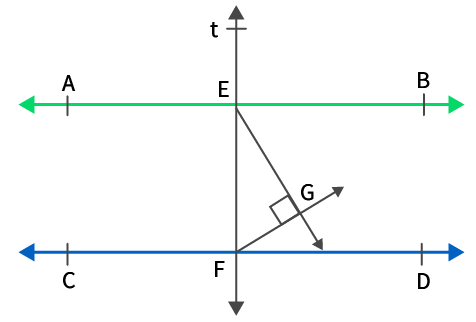Solution:

We know that AB || CD and t is a transversal cutting at points E and F

From the figure we know that ∠BEF and ∠DFE are interior angles

So we get

∠BEF + ∠DFE = 180o

Dividing the entire equation by 2 we get

(1/2)∠BEF + (1/2) ∠DFE = 90o

According to the figure the above equation can further be written as

∠GEF + ∠GFE = 90o ……. (1)

According to the △ GEF

We can write

∠GEF + ∠GFE + ∠EGF = 180o

Based on equation (1) we get

90o + ∠EGF = 180o

On further calculation

∠EGF = 180o – 90o

By subtraction

∠EGF = 90o

Therefore, it is proved that ∠EGF = 90o

19. In the given figure, AB || CD and a transversal t cuts them at E and F respectively. If EP and FQ are the bisectors of ∠AEF and ∠EFD respectively, prove that EP || FQ.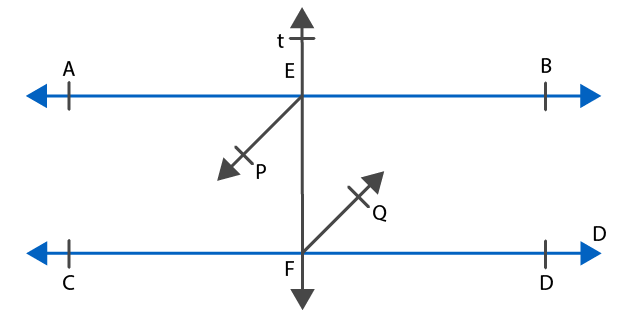Solution:

We know that AB || CD and t is a transversal

From the figure we know that ∠AEF and ∠EFD are alternate angles

So we get

∠AEF = ∠EFD

Dividing both the sides by 2 we get

(1/2) ∠AEF = (1/2) ∠EFD

So we get

∠PEF = ∠EFQ

The alternate interior angles are formed only when the transversal EF cuts both FQ and EP.

Therefore, it is proved that EP || FQ.

20. In the given figure, BA || ED and BC || EF. Show that ∠ABC = ∠DEF.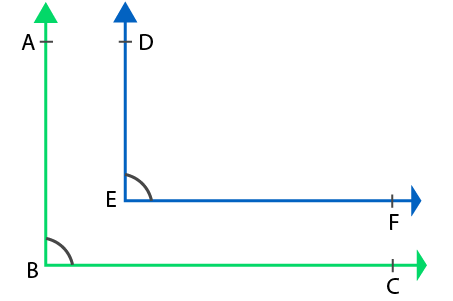Solution:

Extend the line DE to meet the line BE at the point Z.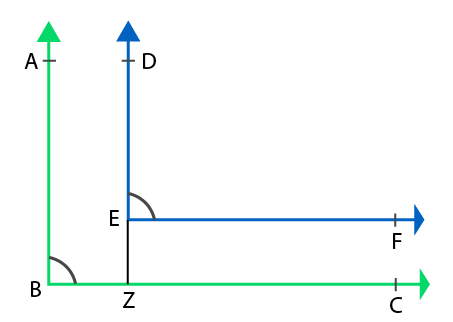We know that AB || DZ and BC is a transversal

From the figure we know that ∠ABC and ∠DZC are corresponding angles

So we get

∠ABC = ∠DZC ….. (1)

We also know that EF || BC and DZ is a transversal

From the figure we know that ∠DZC and ∠DEF are corresponding angles

So we get

∠DZC = ∠DEF …… (2)

Considering both the equation (1) and (2) we get

∠ABC = ∠DEF

Therefore, it is proved that ∠ABC = ∠DEF

21. In the given figure, BA || ED and BC || EF. Show that ∠ABC + ∠DEF = 180o.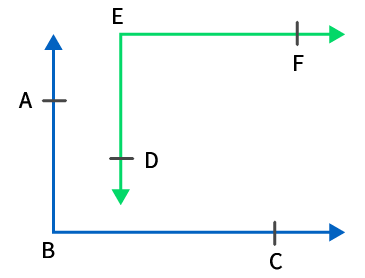Solution:

Extend the line ED to meet the line BC at the point Z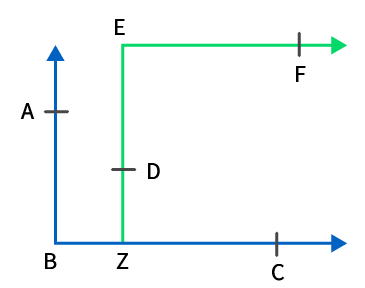We know that AB || EZ and BC is a transversal

From the figure we know that ∠ABZ and ∠EZB are interior angles

So we get

∠ABZ + ∠EZB = 180o

∠ABZ can also be written as ∠ABC

∠ABC + ∠EZB = 180o …… (1)

We know that EF || BC and EZ is a transversal

From the figure we know that ∠BZE and ∠ZEF are alternate angles

So we get

∠BZE = ∠ZEF

∠ZEF can also be written as ∠DEF

∠BZE = ∠DEF ….. (2)

By substituting equation (1) in (2) we get

∠ABC + ∠DEF = 180o

Therefore, it is proved that ∠ABC + ∠DEF = 180o

22. In the given figure, m and n are two plane mirrors perpendicular to each other. Show that the incident ray CA is parallel to the reflected ray BD.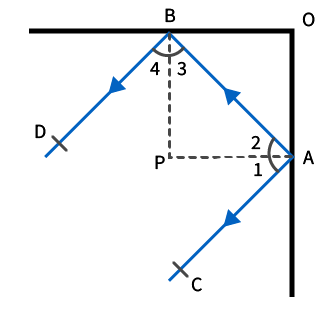Solution:

Construct a line m and n from A and B intersect at P

So we get

OB ⊥ m and OC ⊥ n

So m ⊥ n

We can also write it as

OB ⊥ OC

Since APB is a right angle triangle

We know that ∠APB = 90o

So we can write it as

∠APB = ∠PAB + ∠PBA

By substituting the values

90o = ∠2 + ∠3

We know that angle of incidence is equal to the angle of reflection

So we get

∠1 = ∠2 and ∠4 = ∠3

It can be written as

∠1 + ∠4 = ∠2 + ∠3 = 90o

We can write it as

∠1 + ∠2 + ∠3 + ∠4 = 180o

We know that ∠1 + ∠2 = ∠CAB and ∠3 + ∠4 = ∠ABD

∠CAB + ∠ABD = 180o

According to the diagram ∠CAB and ∠ABD are consecutive interior angles when the transversal AB cuts BD and CA.

Therefore, it is proved that CA || BD.

23. In the figure given below, state which lines are parallel and why?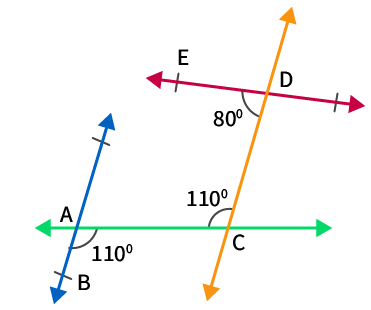Solution:

According to the figure we know that ∠BAC and ∠ACD are alternate angles

So we get

∠BAC = ∠ACD = 110o

We know that ∠BAC and ∠ACD are alternate angles when the transversal AC cuts CD and AB.

Therefore, AB || CD.

24. Two lines are respectively perpendicular to two parallel lines. Show that they are parallel to each other.

Solution: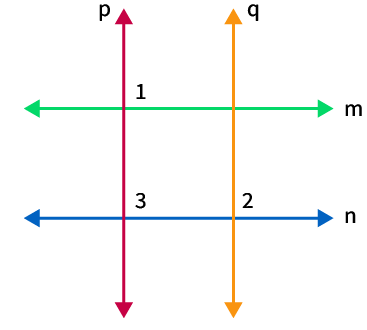According to the figure consider the two parallel lines as m and n

We know that p ⊥ m and q ⊥ n

So we get

∠1 = ∠2 = 90o

We know that m || n and p is a transversal

From the figure we know that ∠1 and ∠3 are corresponding angles

So we get

∠1 = ∠3

We also know that

∠2 = ∠3 = 90o

We know that ∠2 and ∠3 are corresponding angles when the transversal n cuts p and q.

So we get p || q.

Therefore, it is shown that the two lines which are perpendicular to two parallel lines are parallel to each other.

### RS Aggarwal Solutions Class 9 Maths Chapter 7 – Lines and Angles Exercise 7C

RS Aggarwal Solutions Class 9 Maths Chapter 7 Lines and Angles Exercise 7C explains in brief about the results on parallel lines, angles formed when a transversal cuts two lines and corresponding angles axiom.

### Key features of RS Aggarwal Solutions for Class 9 Maths Chapter 7: Lines and Angles Exercise 7C

• The solutions are prepared according to the RS Aggarwal textbook of latest CBSE syllabus.
• The faculty explains the problems in a simple language based on the understanding level of the students.
• PDF of the solutions can be used while solving problems to get their doubts cleared and have clear cut idea about the concepts.
• Helping students to score well in the board exams is the main vision of preparing the solutions.